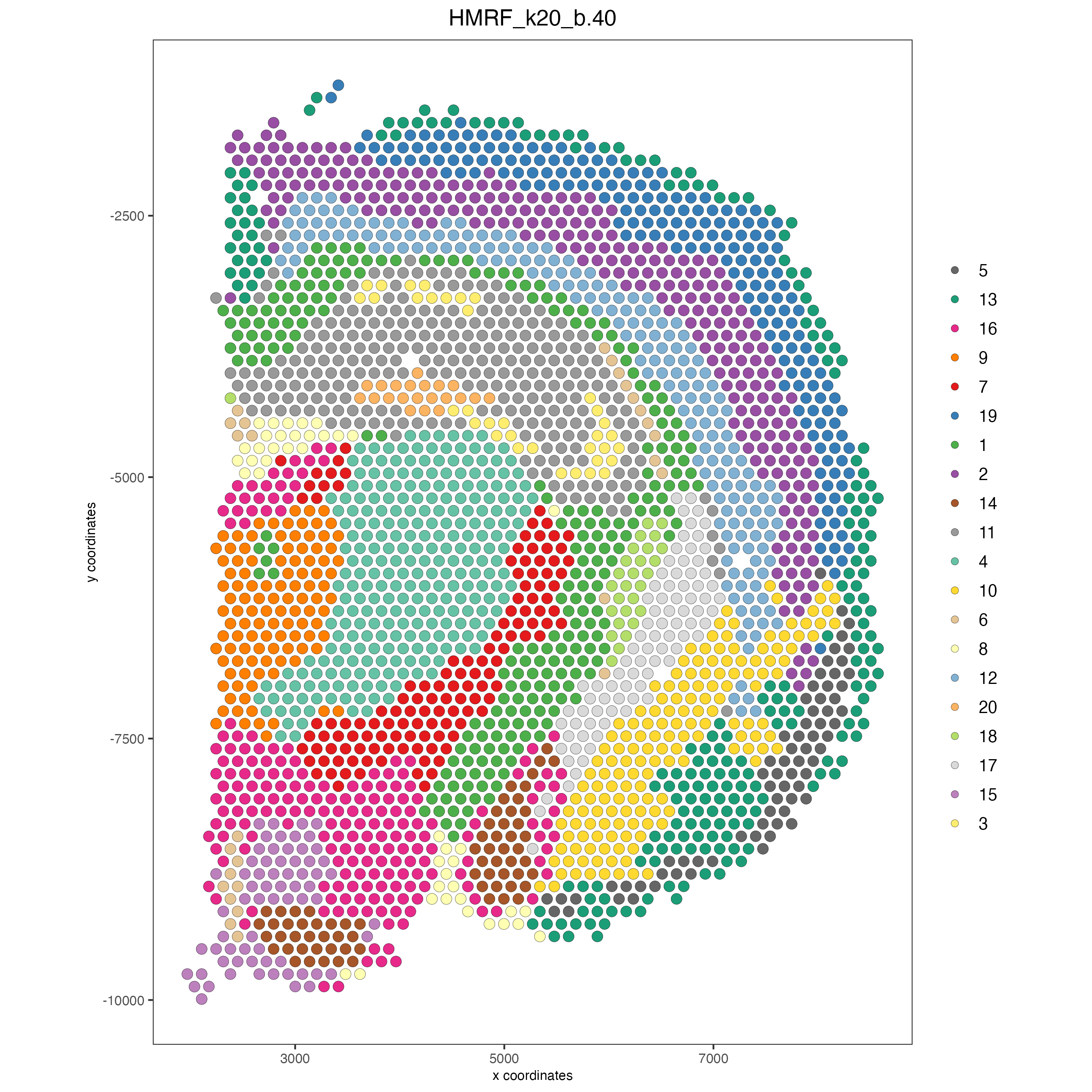# Mouse Visium Brain#

Date:

2022-09-16

```# Ensure Giotto Suite is installed.
if(!"Giotto" %in% installed.packages()) {
devtools::install_github("drieslab/Giotto@suite")
}

# Ensure GiottoData, a small, helper module for tutorials, is installed.
if(!"GiottoData" %in% installed.packages()) {
devtools::install_github("drieslab/GiottoData")
}
library(Giotto)
# Ensure the Python environment for Giotto has been installed.
genv_exists = checkGiottoEnvironment()
if(!genv_exists){
# The following command need only be run once to install the Giotto environment.
installGiottoEnvironment()
}
```

## Set up Giotto Environment#

```library(Giotto)
library(GiottoData)

# 1. set working directory
results_folder = 'path/to/result'

# Optional: Specify a path to a Python executable within a conda or miniconda
# environment. If set to NULL (default), the Python executable within the previously
# installed Giotto environment will be used.
my_python_path = NULL # alternatively, "/local/python/path/python" if desired.

# 3. Create Giotto Instructions
instrs = createGiottoInstructions(save_dir = results_folder,
save_plot = TRUE,
show_plot = FALSE,
python_path = my_python_path)
```

## Dataset explanation#

10X genomics recently launched a new platform to obtain spatial expression data using a Visium Spatial Gene Expression slide.

The Visium brain data to run this tutorial can be found here

## Part 1: Create Giotto Visium Object and visualize#

```## provide path to visium folder
data_path = '/path/to/Brain_data/'

## directly from visium folder
visium_brain = createGiottoVisiumObject(visium_dir = data_path,
expr_data = 'raw',
png_name = 'tissue_lowres_image.png',
gene_column_index = 2,
instructions = instrs)

## show associated images with giotto object
showGiottoImageNames(visium_brain) # "image" is the default name

## show plot
spatPlot2D(gobject = visium_brain, cell_color = 'in_tissue', point_size = 2,
cell_color_code = c('0' = 'lightgrey', '1' = 'blue'),
show_image = T, image_name = 'image')
```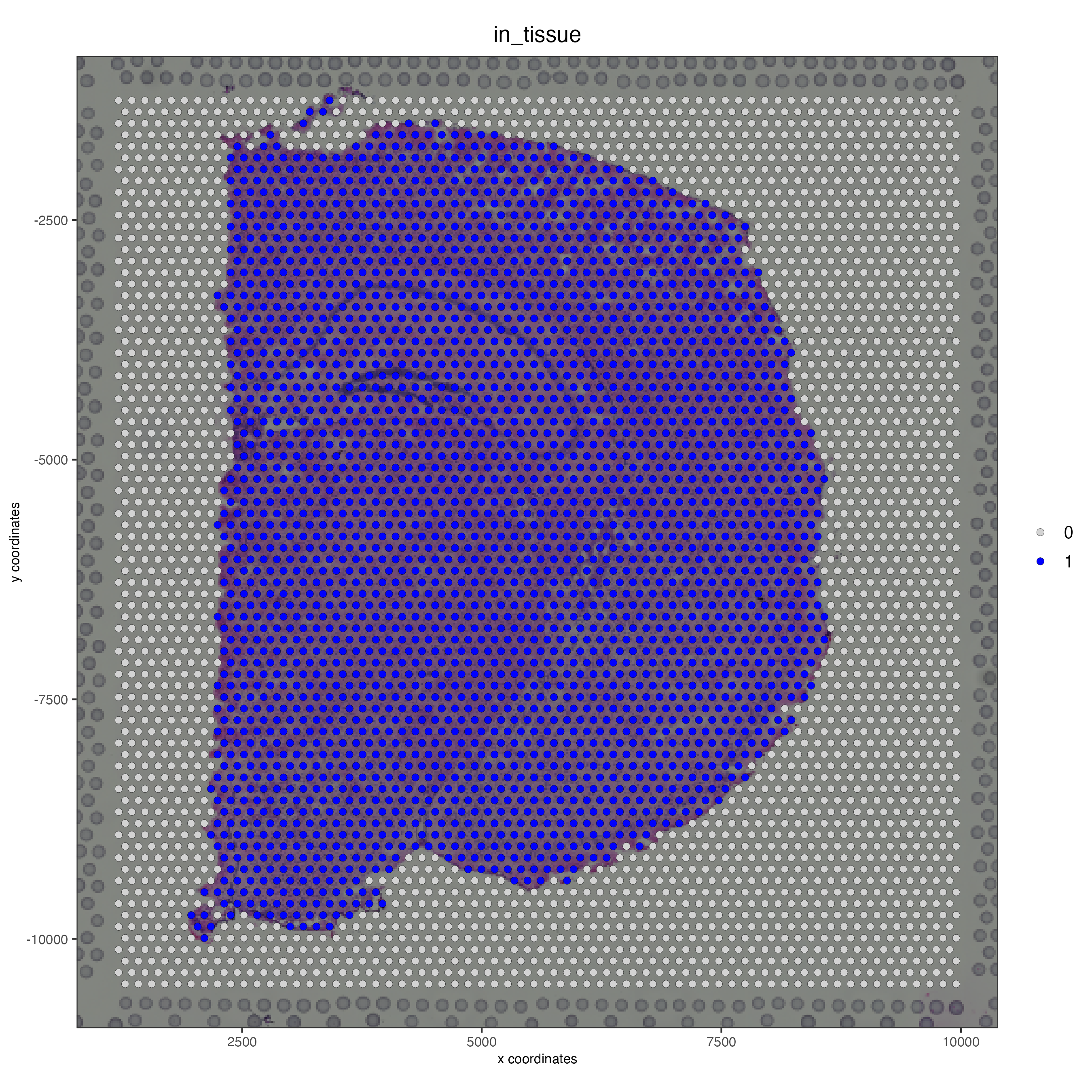## Part 2: Process Giotto Visium Object#

```## subset on spots that were covered by tissue
visium_brain = subsetGiotto(visium_brain, cell_ids = in_tissue_barcodes)

## filter
visium_brain <- filterGiotto(gobject = visium_brain,
expression_threshold = 1,
feat_det_in_min_cells = 50,
min_det_feats_per_cell = 1000,
expression_values = c('raw'),
verbose = T)

## normalize
visium_brain <- normalizeGiotto(gobject = visium_brain, scalefactor = 6000, verbose = T)

## add gene & cell statistics

## visualize
spatPlot2D(gobject = visium_brain, show_image = T, point_alpha = 0.7,
cell_color = 'nr_feats', color_as_factor = F)
```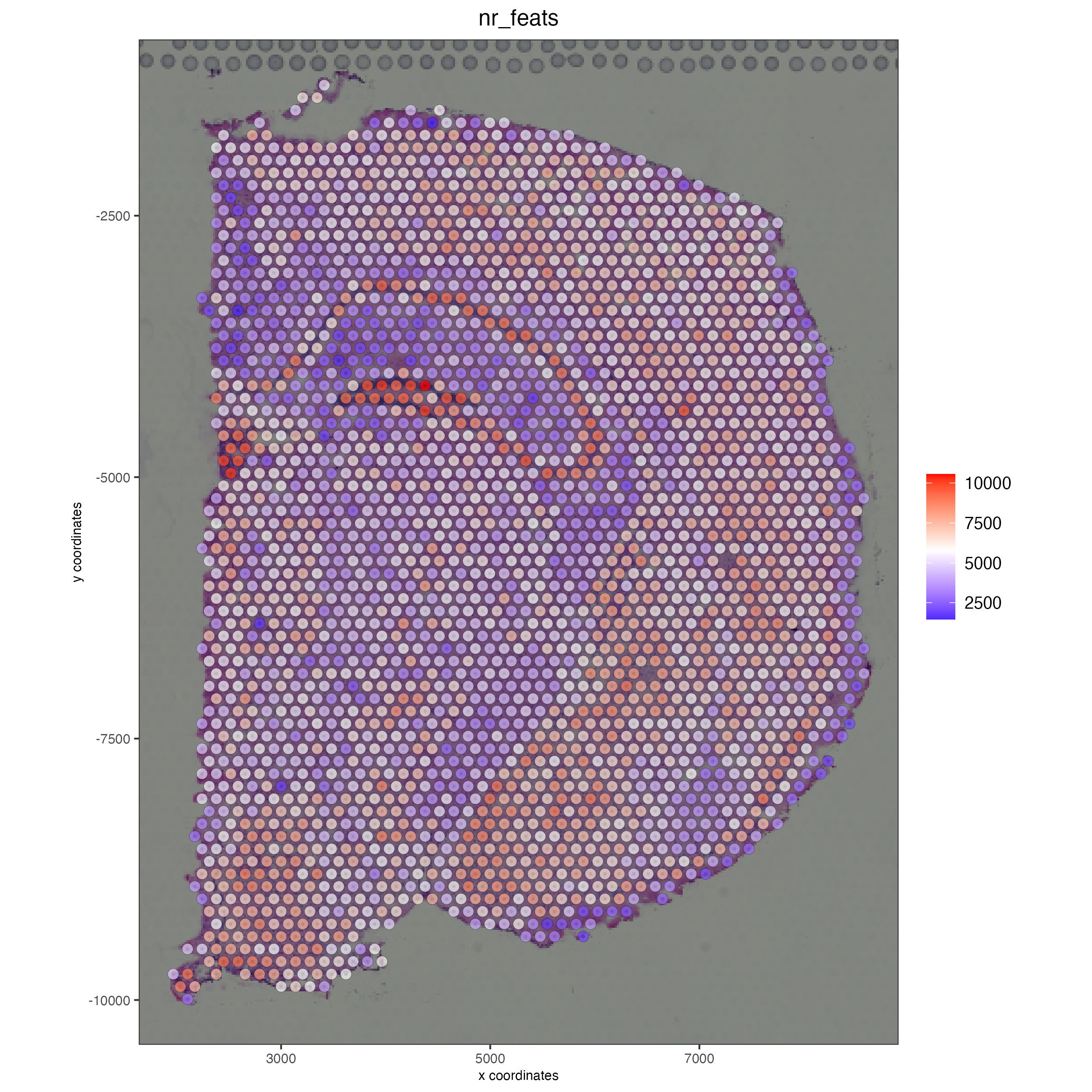## Part 3: Dimention Reduction#

```## highly variable features / genes (HVF)
visium_brain <- calculateHVF(gobject = visium_brain, save_plot = TRUE)
```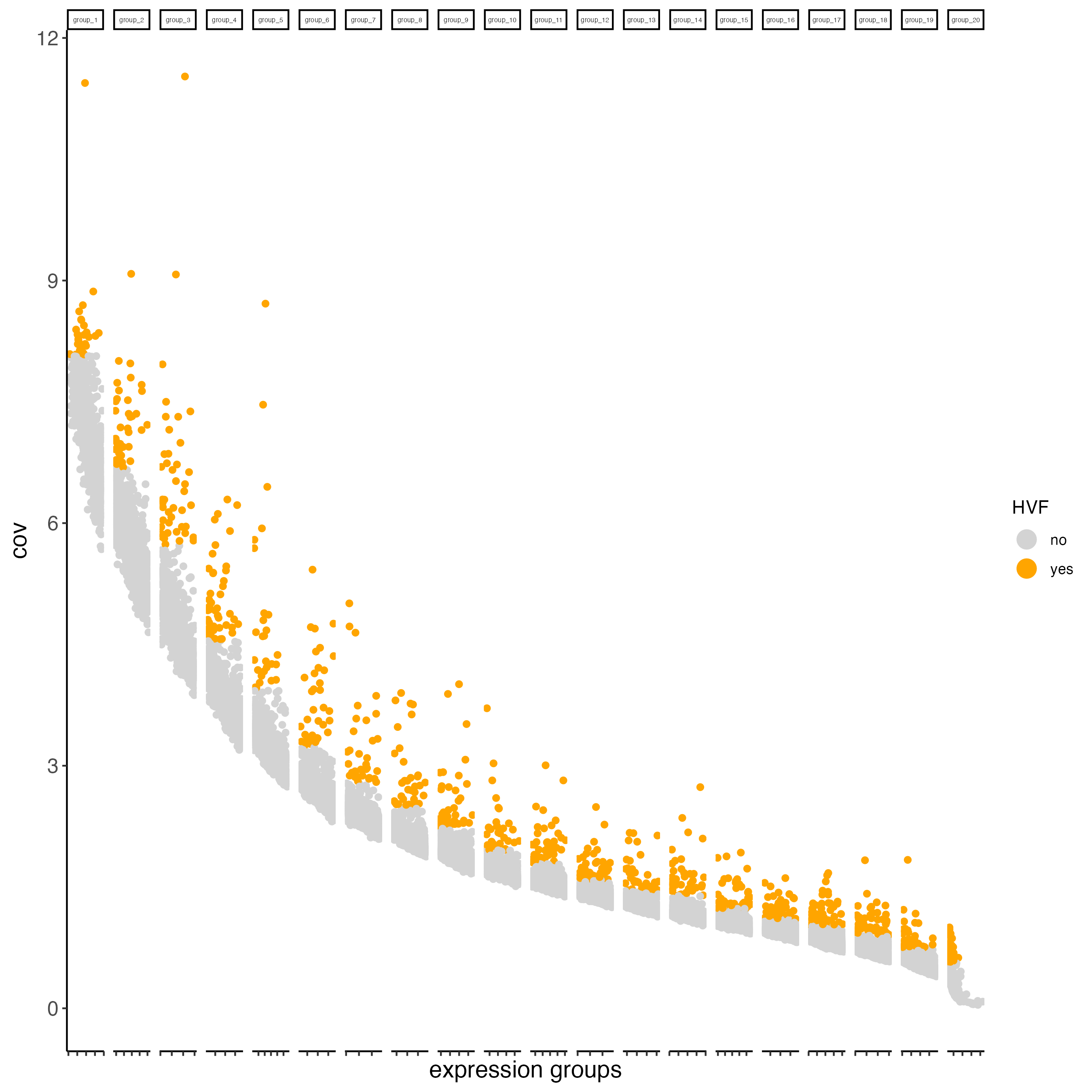```## run PCA on expression values (default)
featgenes = gene_metadata[hvf == 'yes' & perc_cells > 3 & mean_expr_det > 0.4]\$feat_ID

## run PCA on expression values (default)
visium_brain <- runPCA(gobject = visium_brain,
feats_to_use = featgenes)

screePlot(visium_brain, ncp = 30)
```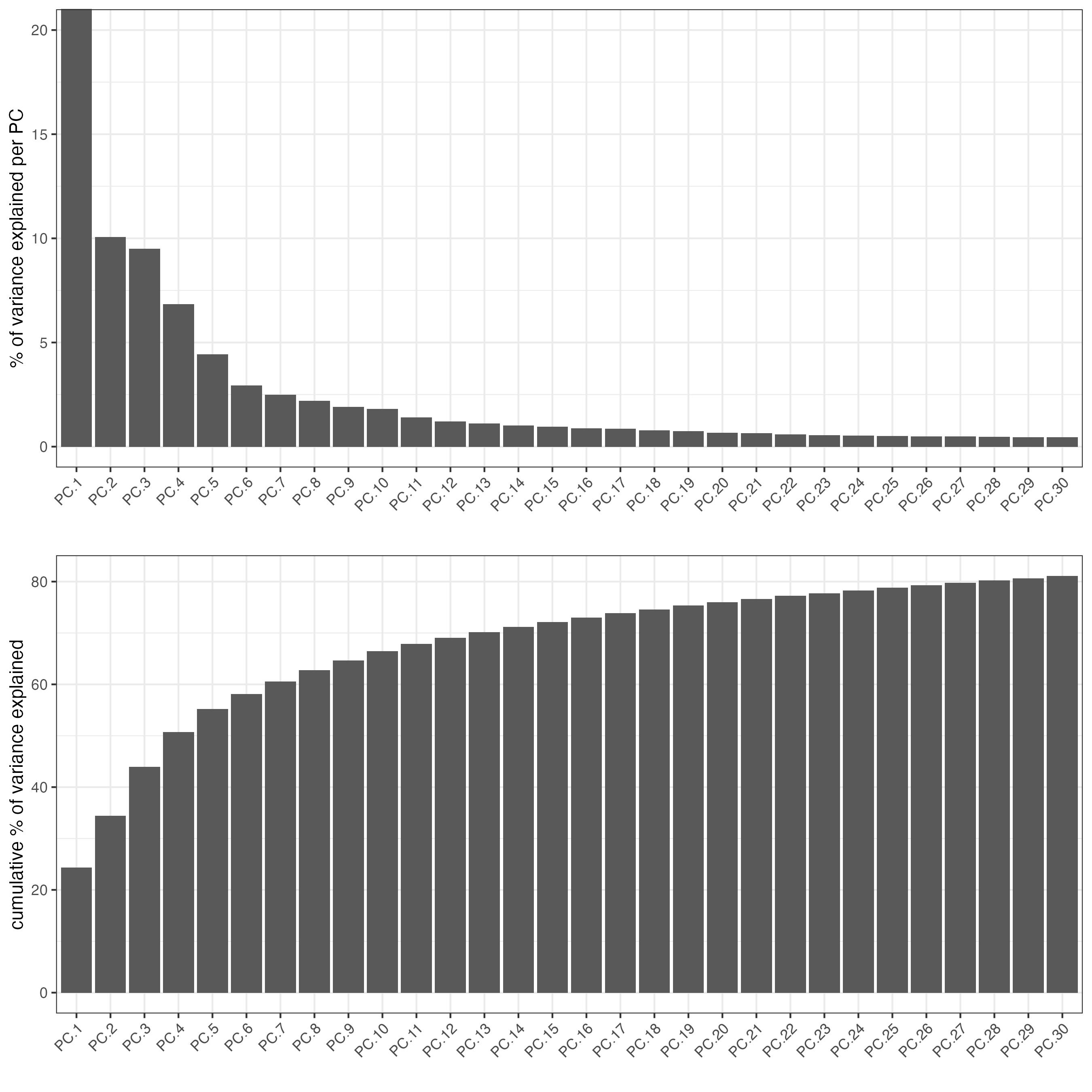```dimPlot2D(gobject = visium_brain,dim_reduction_to_use = "pca")
```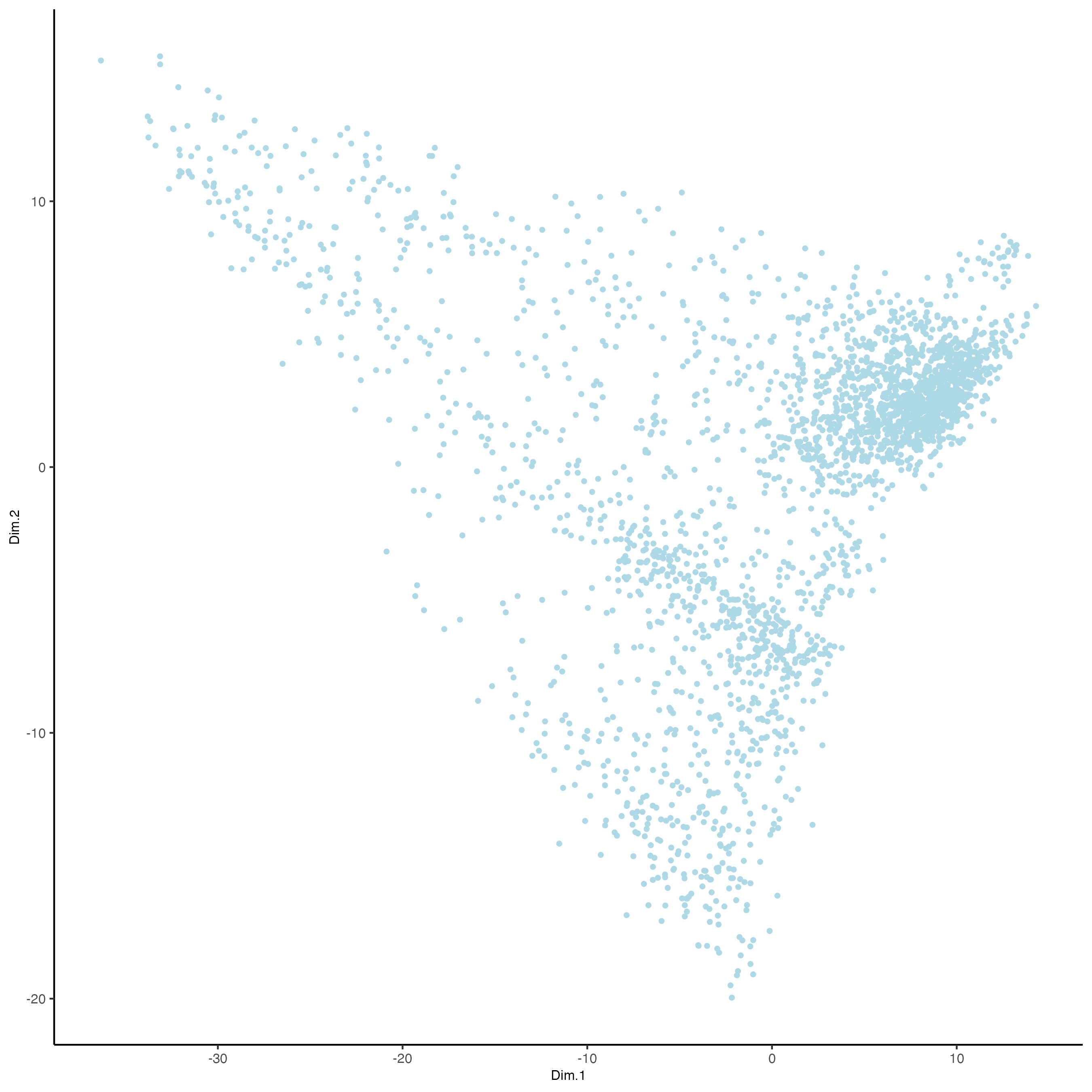```## run UMAP and tSNE on PCA space (default)
visium_brain <- runUMAP(visium_brain, dimensions_to_use = 1:10)
plotUMAP(gobject = visium_brain)
```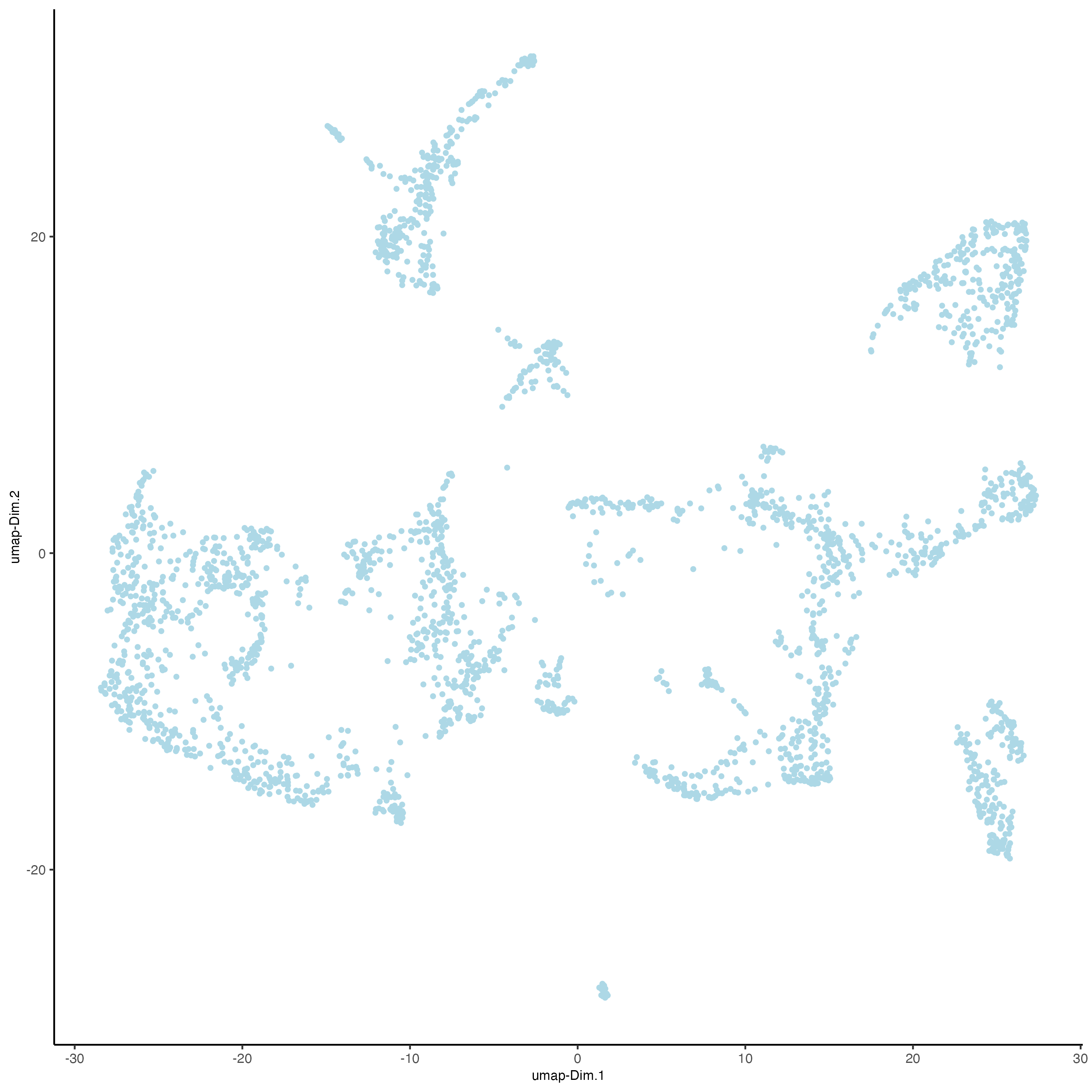```visium_brain <- runtSNE(visium_brain, dimensions_to_use = 1:10)
plotTSNE(gobject = visium_brain)
```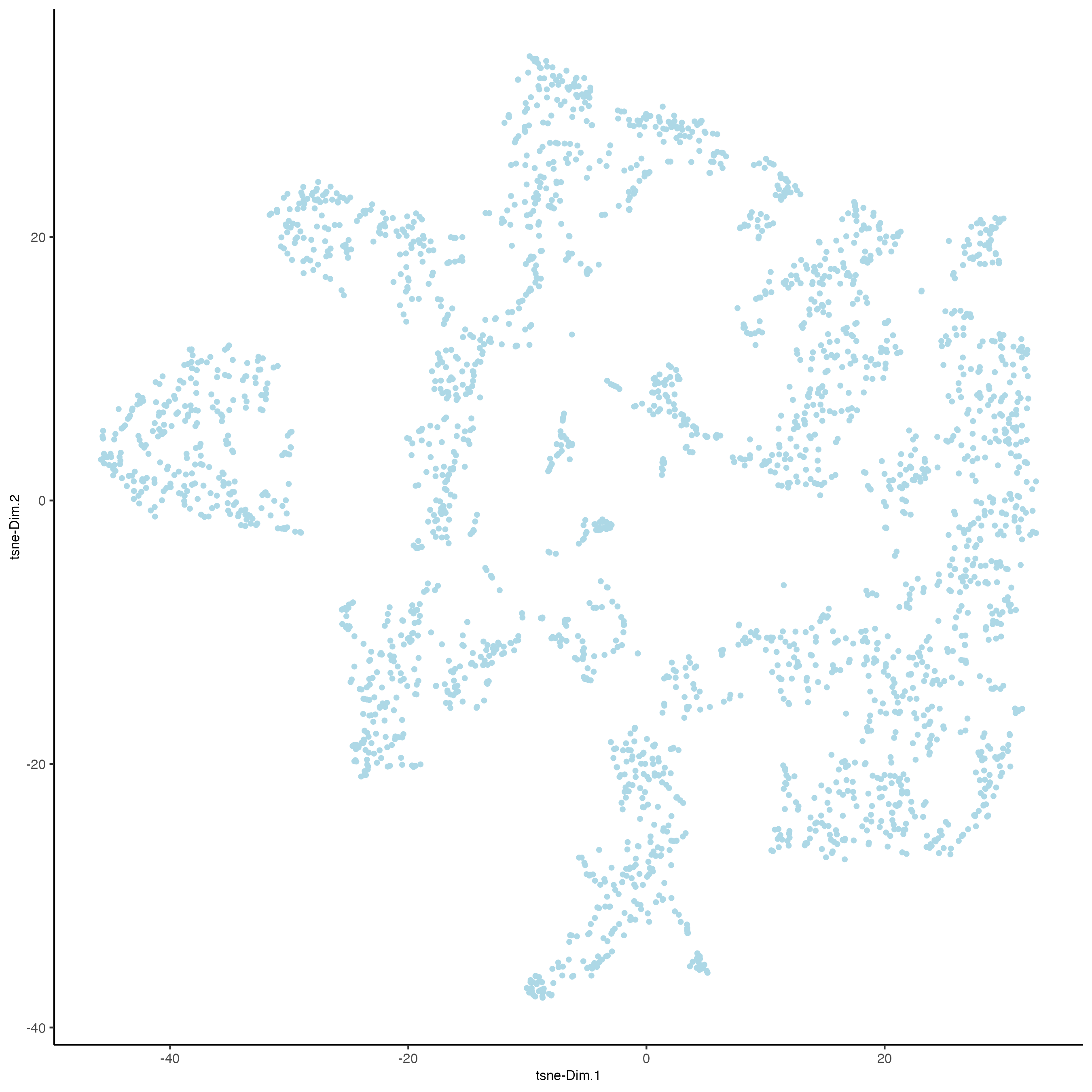## Part 4: Cluster#

```## sNN network (default)
visium_brain <- createNearestNetwork(gobject = visium_brain, dimensions_to_use = 1:10, k = 15)

## Leiden clustering
visium_brain <- doLeidenCluster(gobject = visium_brain, resolution = 0.4, n_iterations = 1000)

plotUMAP(gobject = visium_brain,
cell_color = 'leiden_clus', show_NN_network = T, point_size = 2.5)
```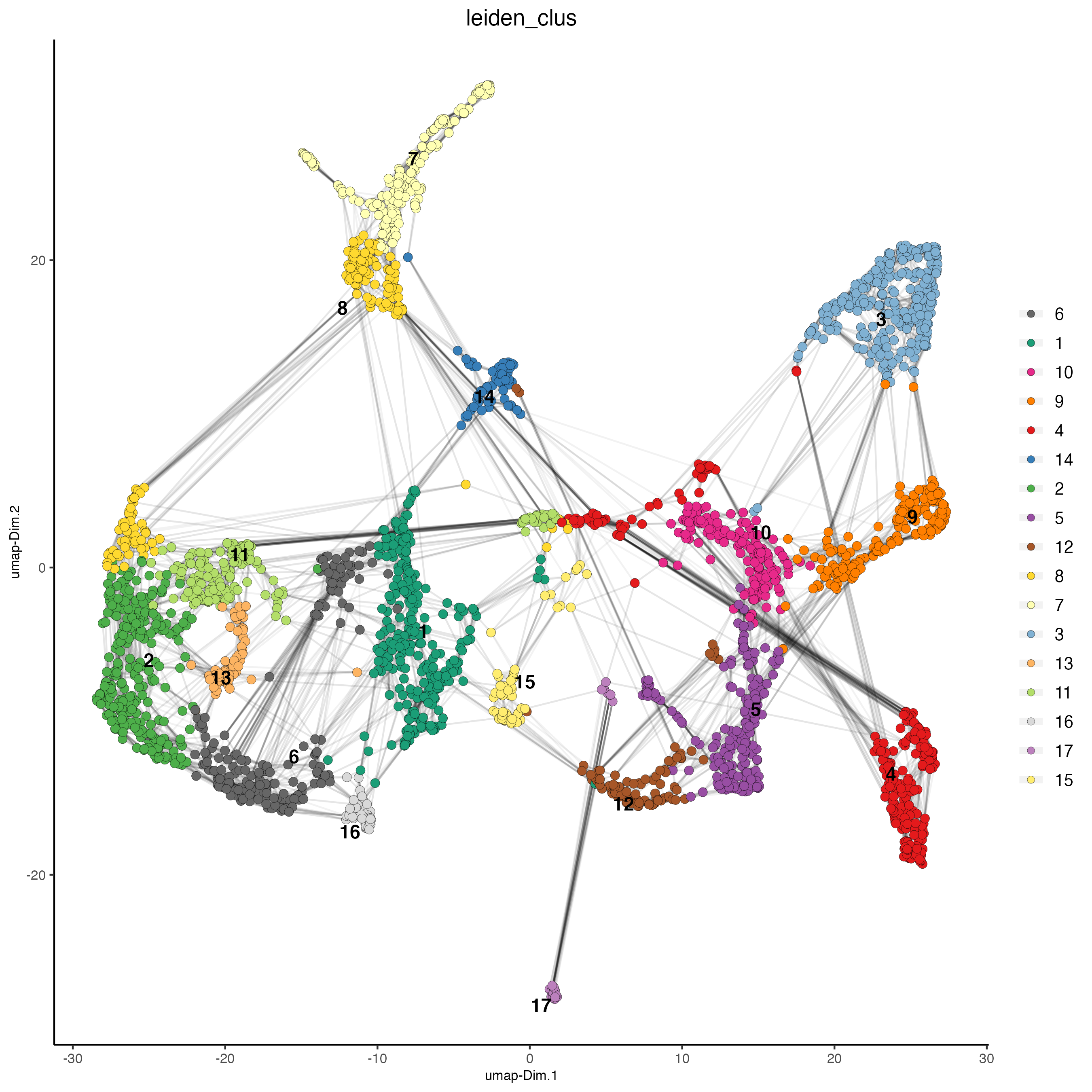```# spatial and dimension plots
spatDimPlot(gobject = visium_brain, cell_color = 'leiden_clus',
dim_point_size = 2, spat_point_size = 2.5)
```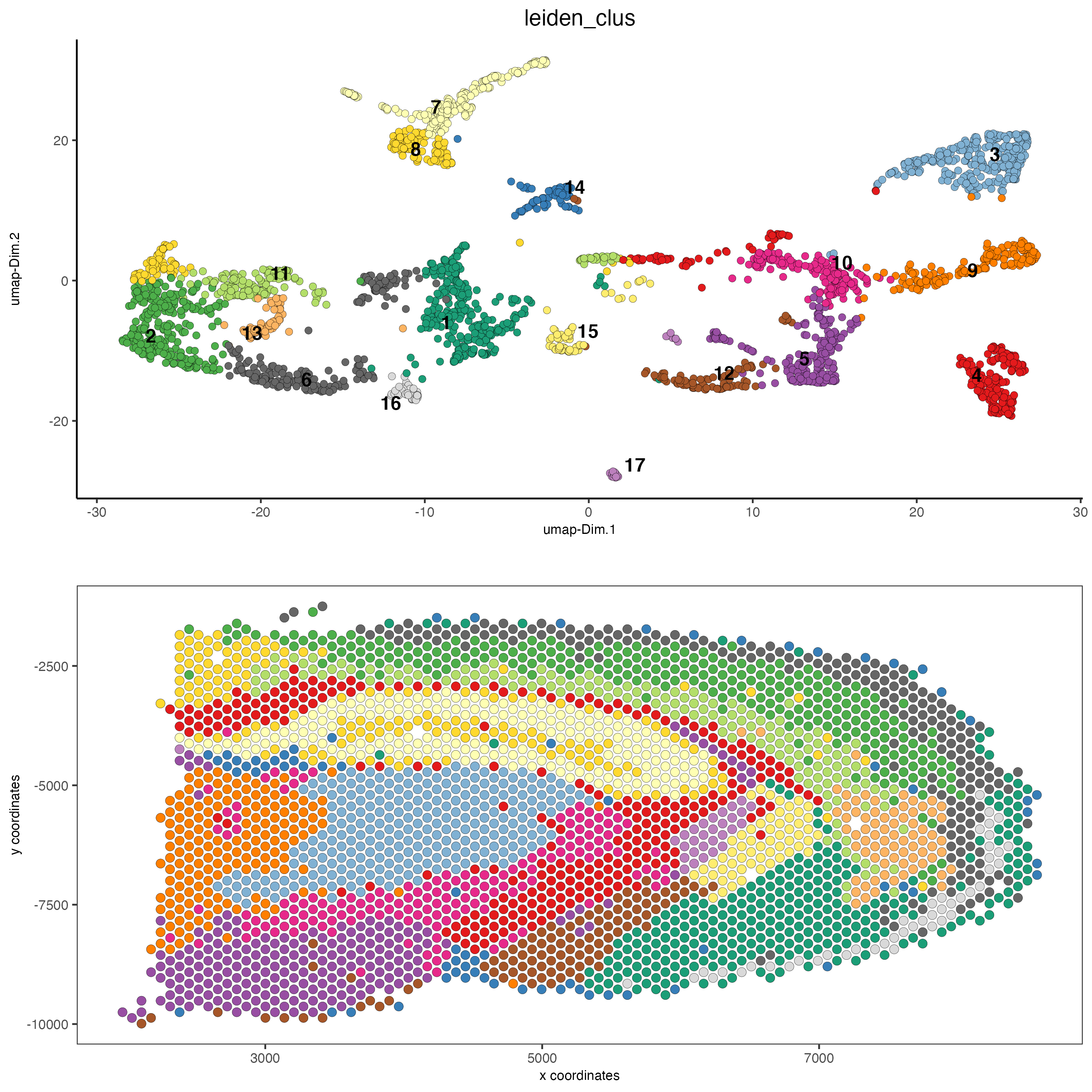```spatDimPlot(gobject = visium_brain, cell_color = 'nr_feats', color_as_factor = F,
dim_point_size = 2, spat_point_size = 2.5)
```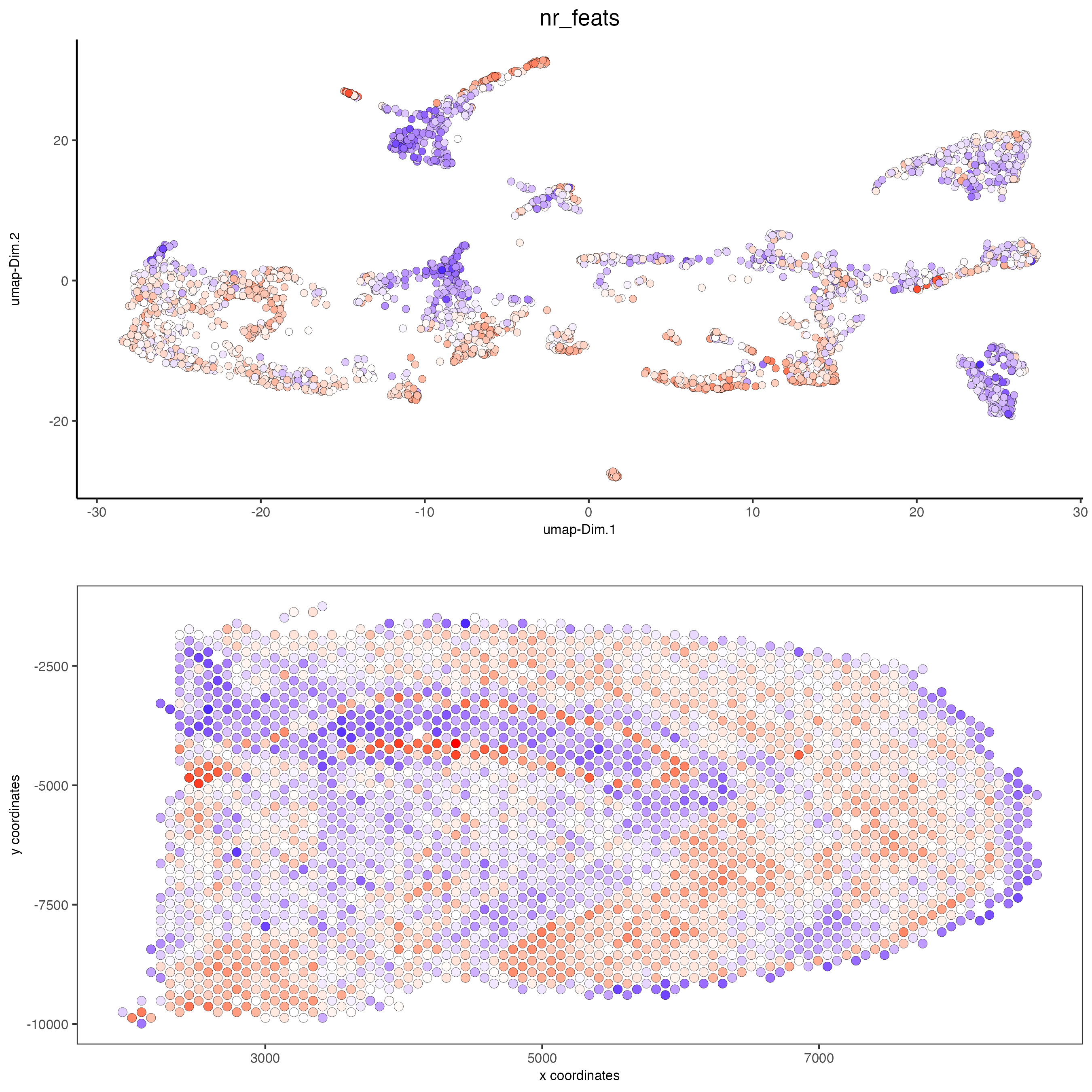```# dimension plots grouped by cluster
spatPlot2D(visium_brain, cell_color = 'leiden_clus',
coord_fix_ratio = 1)
```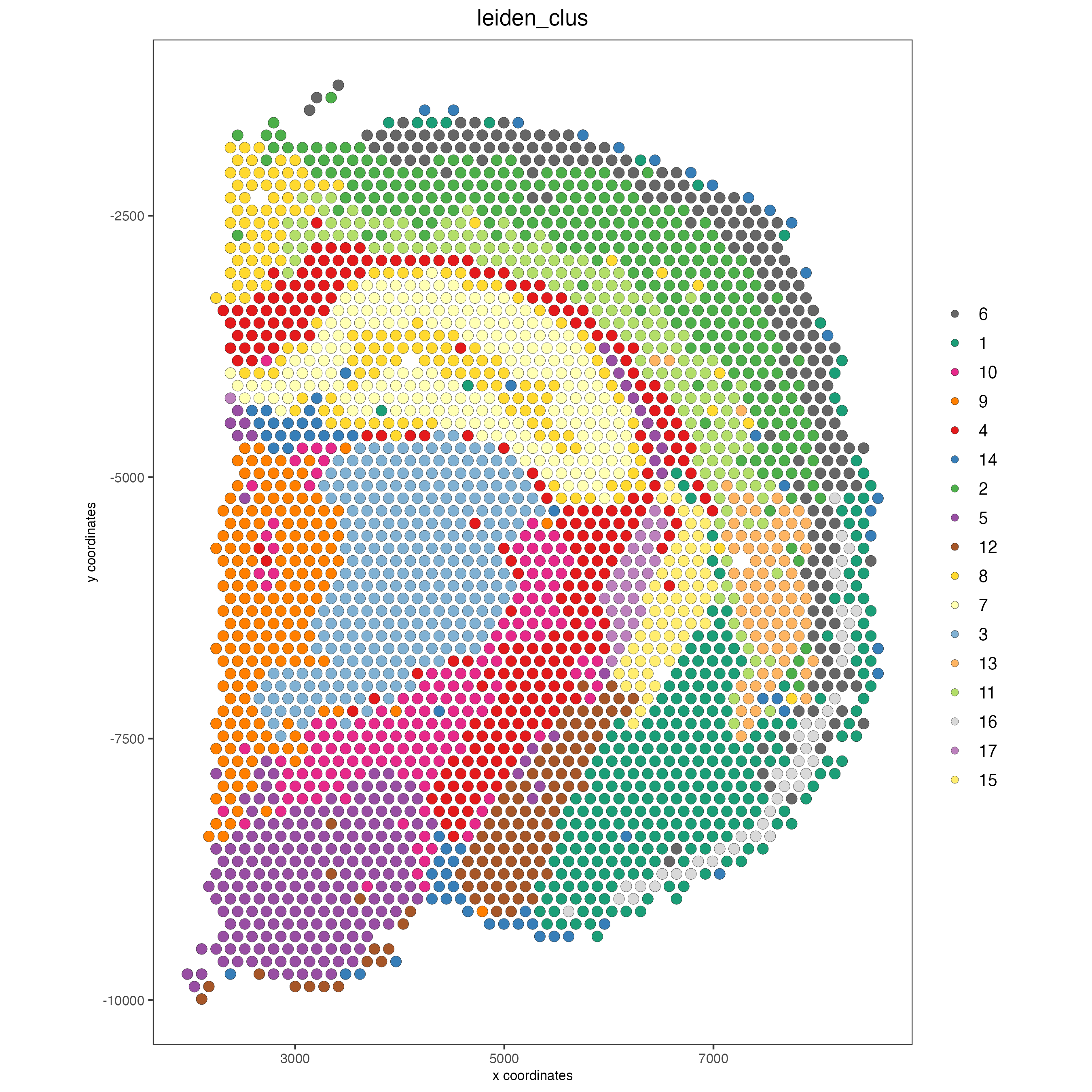Plot with group by:

```spatPlot2D(visium_brain, cell_color = 'leiden_clus',
group_by = 'leiden_clus', coord_fix_ratio = 1,
cow_n_col = 6, show_legend = F,
save_param = list(base_width = 14, base_height = 14))
```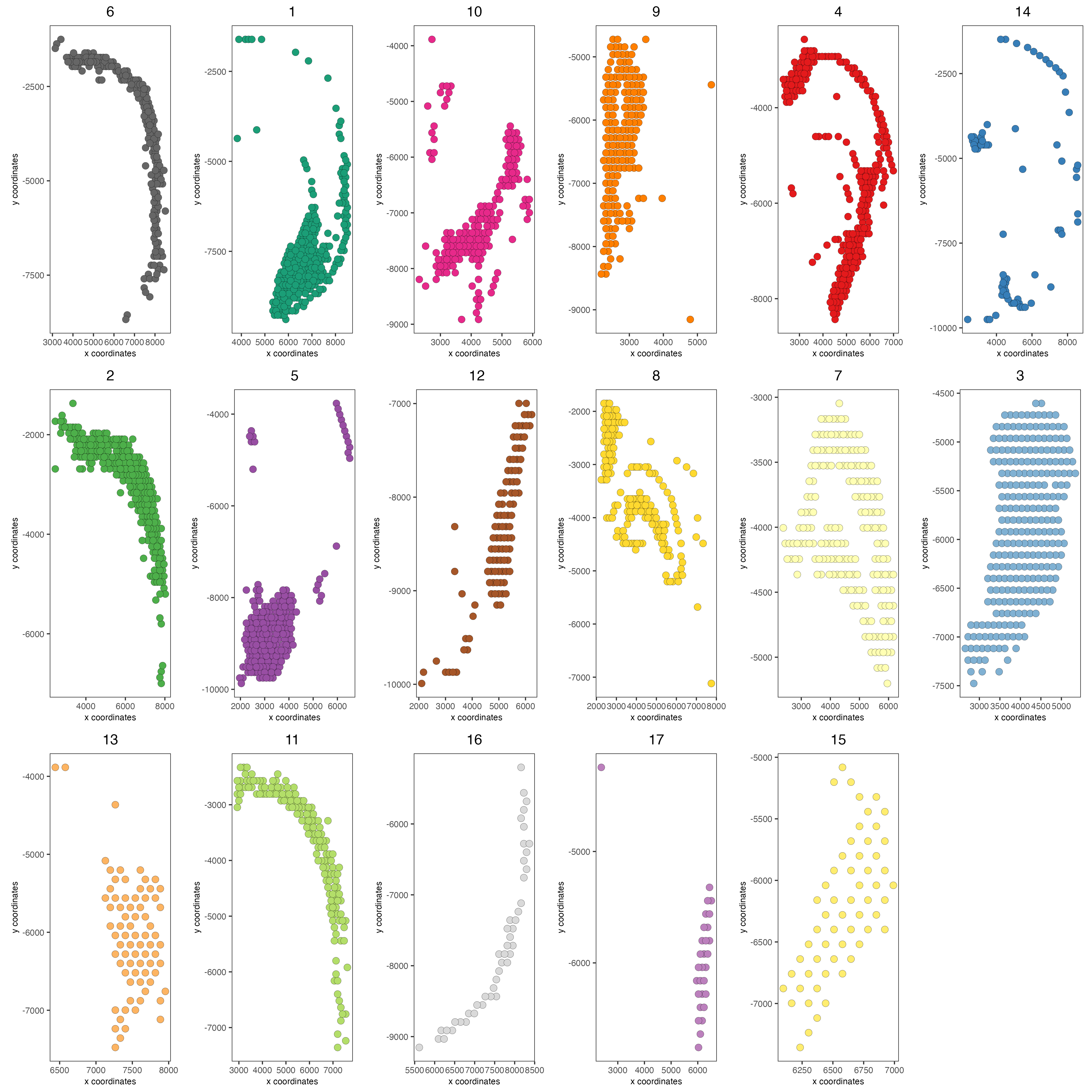Highlight one or more groups:

```spatPlot2D(visium_brain, cell_color = 'leiden_clus',
select_cell_groups = '8', coord_fix_ratio = 1, show_other_cells = TRUE,
cell_color_code = c('8' = 'red'), other_cell_color = "grey", other_point_size = 1.5,
save_param = list(base_width = 7, base_height = 7))
```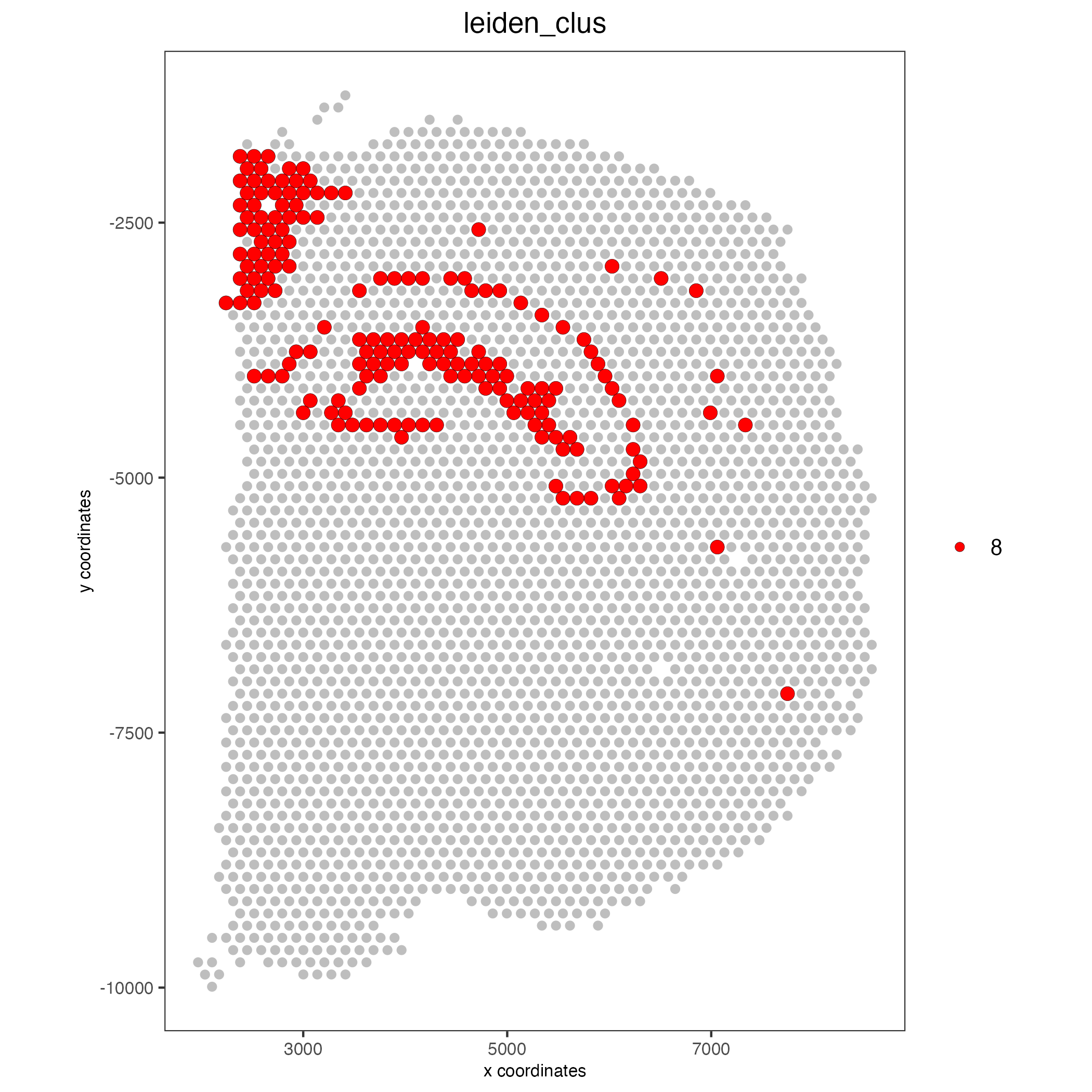## Part 5: subset data#

```# create and show subset
DG_subset = subsetGiottoLocs(visium_brain,
x_max = 6500, x_min = 3000,
y_max = -2500, y_min = -5500,
return_gobject = TRUE)

spatDimPlot(gobject = DG_subset,
cell_color = 'leiden_clus', spat_point_size = 5)
```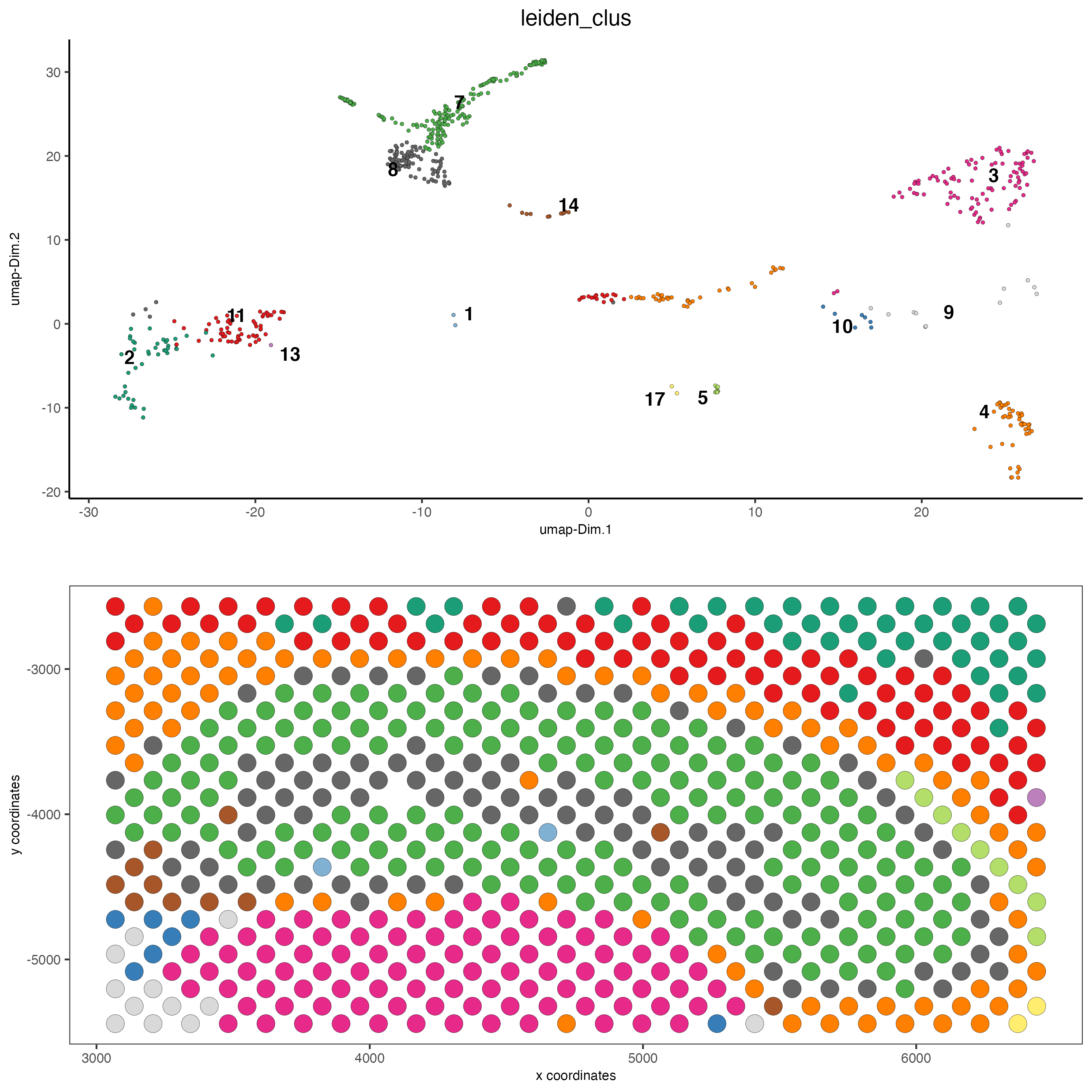## Part 6: marker gene detection for clusters#

```## ------------------ ##
## Gini markers
gini_markers_subclusters = findMarkers_one_vs_all(gobject = visium_brain,
method = 'gini',
expression_values = 'normalized',
cluster_column = 'leiden_clus',
min_feats = 20,
min_expr_gini_score = 0.5,
min_det_gini_score = 0.5)
topgenes_gini = gini_markers_subclusters[, head(.SD, 2), by = 'cluster']\$feats

# violinplot
violinPlot(visium_brain, feats = unique(topgenes_gini), cluster_column = 'leiden_clus',
strip_text = 8, strip_position = 'right',
save_param = list(base_width = 5, base_height = 10))
```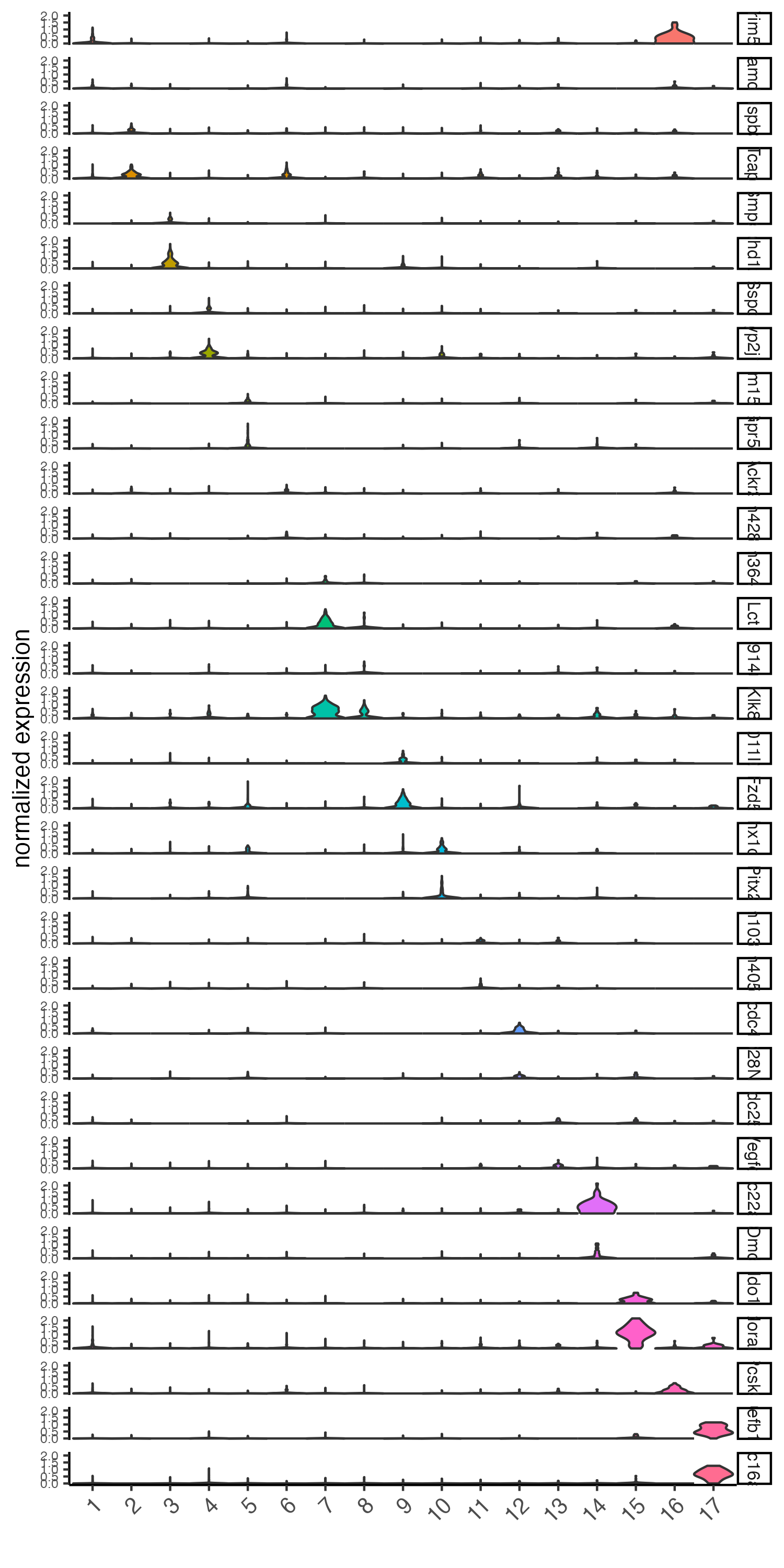```# cluster heatmap
x_text_size = 10, y_text_size = 10)
```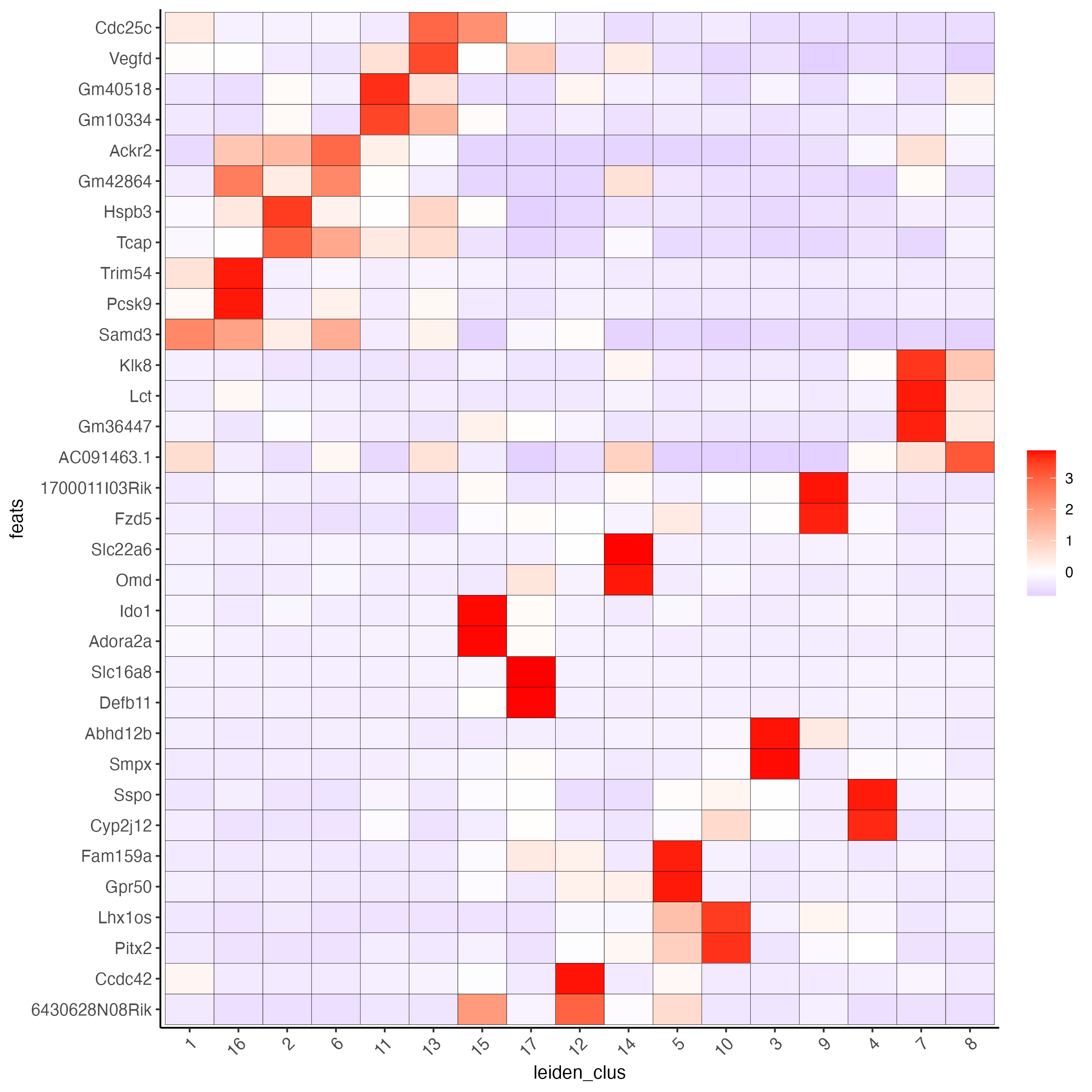```# umap plots
dimFeatPlot2D(visium_brain, expression_values = 'scaled',
feats = gini_markers_subclusters[, head(.SD, 1), by = 'cluster']\$feats,
cow_n_col = 4, point_size = 0.75,
save_param = list(base_width = 8, base_height = 8))
```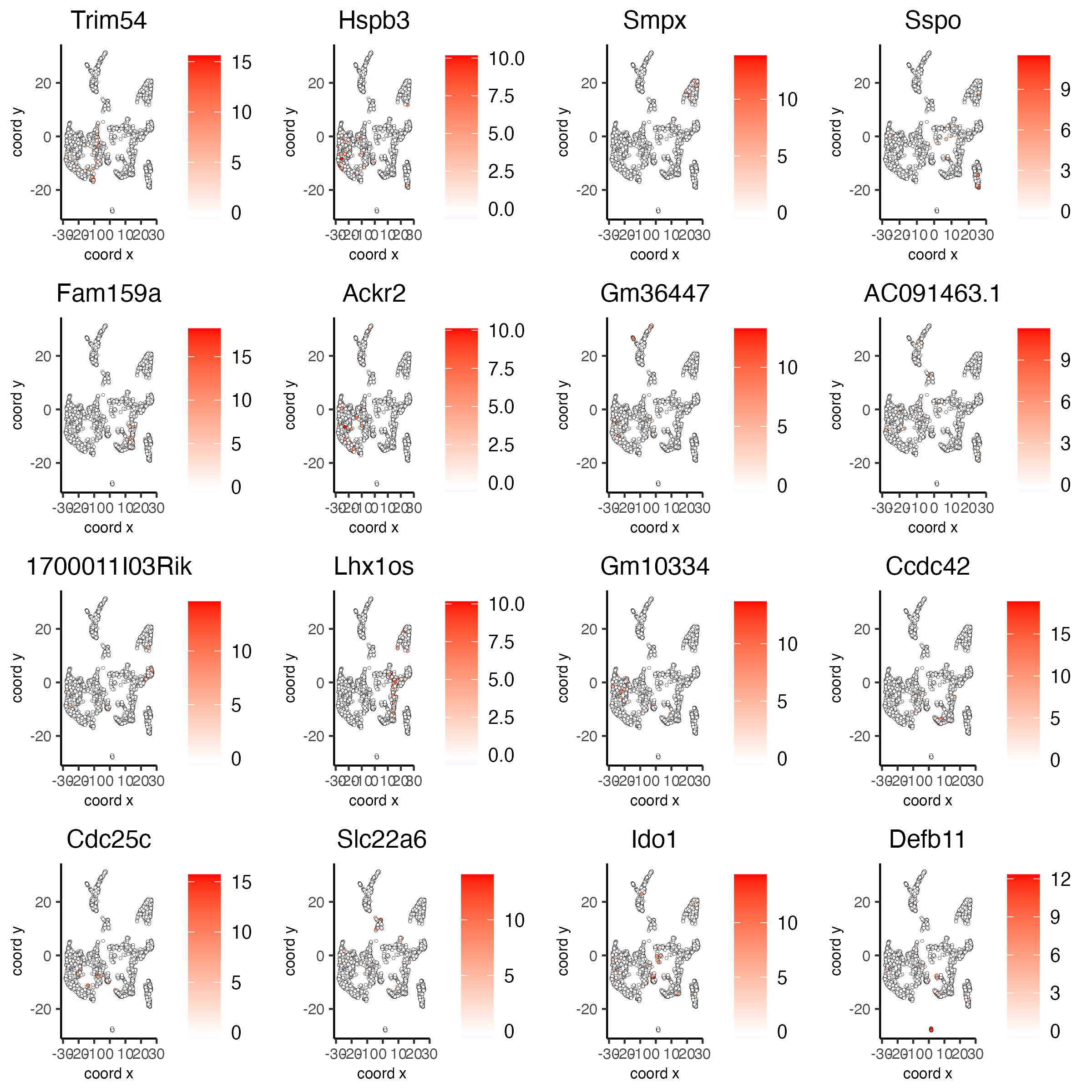```## ------------------ ##
# Scran Markers
scran_markers_subclusters = findMarkers_one_vs_all(gobject = visium_brain,
method = 'scran',
expression_values = 'normalized',
cluster_column = 'leiden_clus')
topgenes_scran = scran_markers_subclusters[, head(.SD, 2), by = 'cluster']\$feats

# violinplot
violinPlot(visium_brain, feats = unique(topgenes_scran), cluster_column = 'leiden_clus',
strip_text = 10, strip_position = 'right',
save_param = list(base_width = 5))
```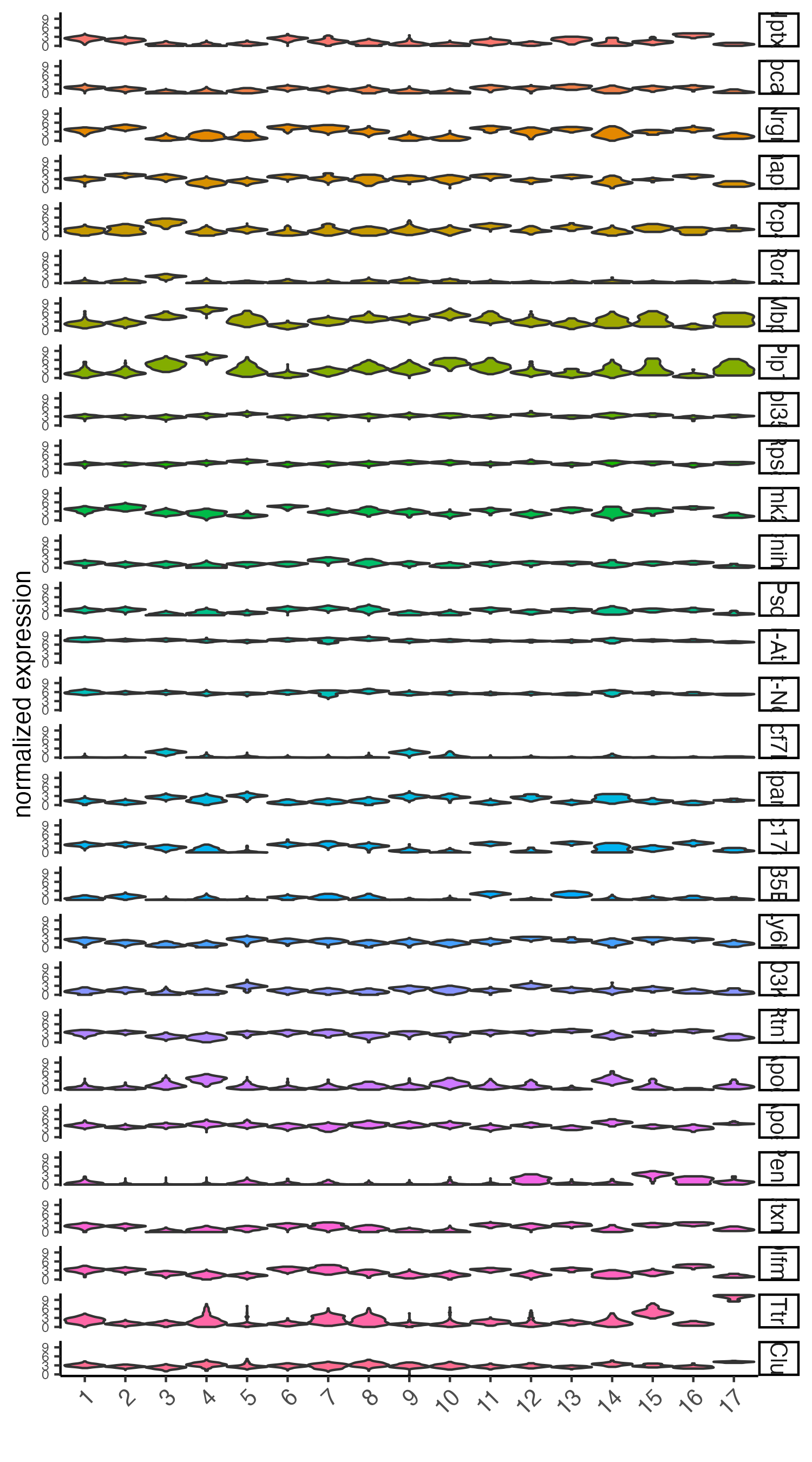```# cluster heatmap
```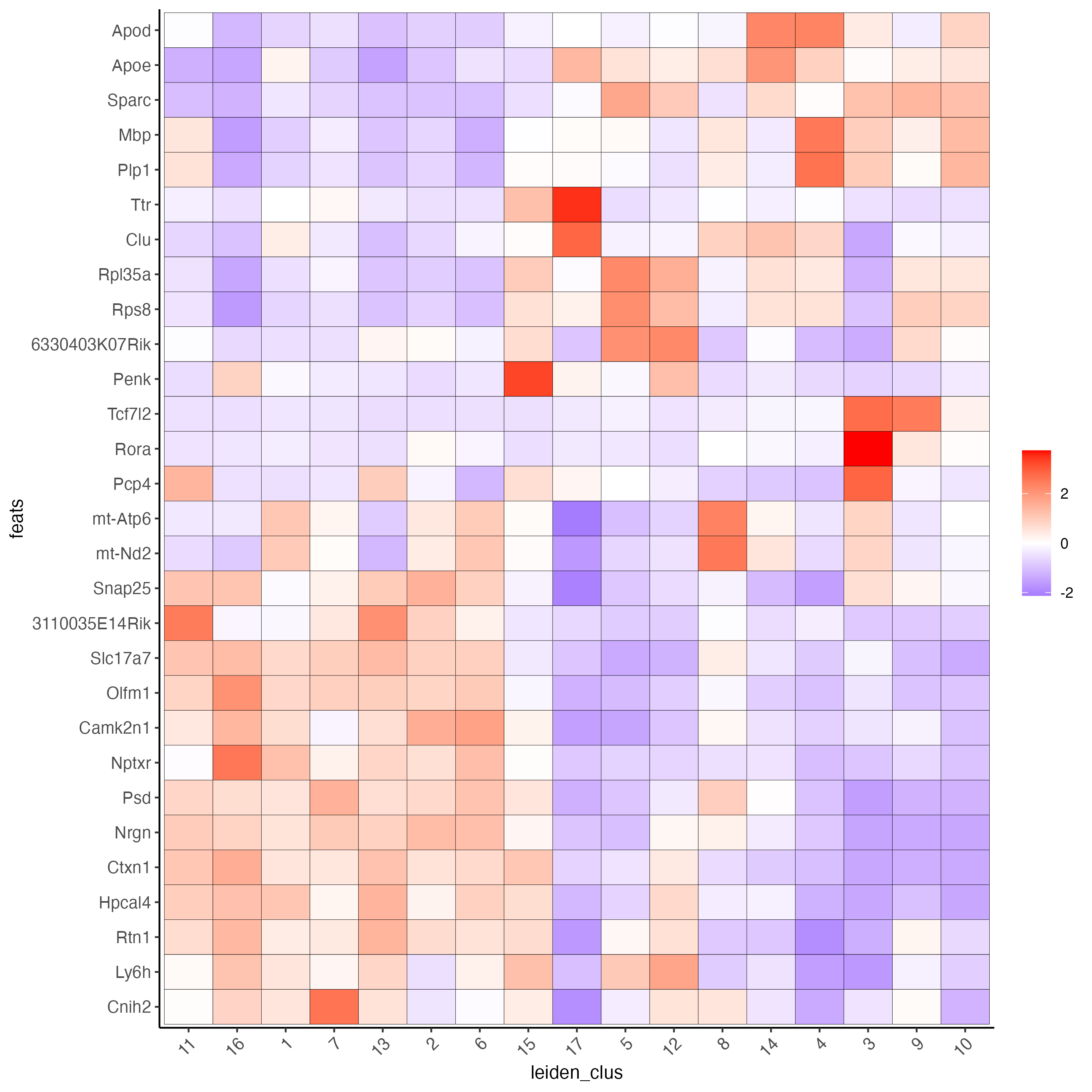```# umap plots
dimFeatPlot2D(visium_brain, expression_values = 'scaled',
feats = scran_markers_subclusters[, head(.SD, 1), by = 'cluster']\$feats,
cow_n_col = 3, point_size = 1,
save_param = list(base_width = 8, base_height = 8))
```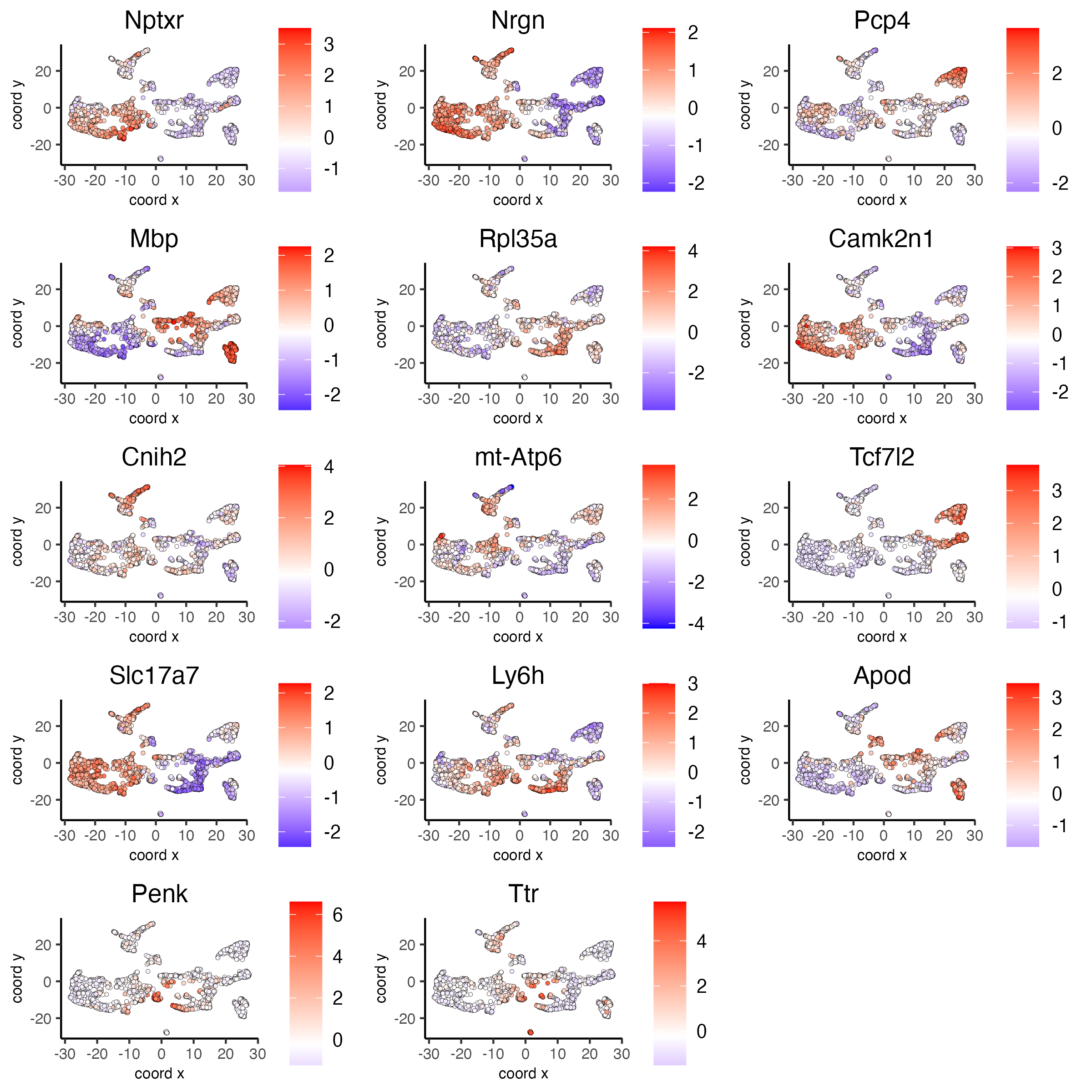## Part 7: Cell type enrichment#

Visium spatial transcriptomics does not provide single-cell resolution, making cell type annotation a harder problem. Giotto provides several ways to calculate enrichment of specific cell-type signature gene lists:
- PAGE
- hypergeometric test
- Rank
- DWLS Deconvolution Corresponded Single cell dataset can be generated from here. Giotto_SC is processed from the downsampled Loom file and can also be downloaded from getSpatialDataset.
```# download data to results directory ####
# if wget is installed, set method = 'wget'
# if you run into authentication issues with wget, then add " extra = '--no-check-certificate' "
getSpatialDataset(dataset = 'scRNA_mouse_brain', directory = results_folder)

sc_expression = paste0(results_folder, "/brain_sc_expression_matrix.txt.gz")

giotto_SC <- createGiottoObject(
expression = sc_expression,
instructions = instrs
)

giotto_SC<- normalizeGiotto(giotto_SC)
```

### 7.1 PAGE enrichment#

```# Create PAGE matrix
# PAGE matrix should be a binary matrix with each row represent a gene marker and each column represent a cell type
# There are several ways to create PAGE matrix
# 1.1 create binary matrix of cell signature genes
# small example #
gran_markers = c("Nr3c2", "Gabra5", "Tubgcp2", "Ahcyl2",
"Islr2", "Rasl10a", "Tmem114", "Bhlhe22",
"Ntf3", "C1ql2")

oligo_markers = c("Efhd1", "H2-Ab1", "Enpp6", "Ninj2",
"Bmp4", "Tnr", "Hapln2", "Neu4",
"Wfdc18", "Ccp110")

di_mesench_markers = c("Cartpt", "Scn1a", "Lypd6b",  "Drd5",
"Gpr88", "Plcxd2", "Cpne7", "Pou4f1",
"Ctxn2", "Wnt4")

PAGE_matrix_1 = makeSignMatrixPAGE(sign_names = c('Granule_neurons',
'Oligo_dendrocytes',
'di_mesenchephalon'),
sign_list = list(gran_markers,
oligo_markers,
di_mesench_markers))

# ----

# 1.2 [shortcut] fully pre-prepared matrix for all cell types
sign_matrix_path = system.file("extdata", "sig_matrix.txt", package = 'Giotto')
PAGE_matrix_2 = as.matrix(brain_sc_markers[,-1])
rownames(PAGE_matrix_2) = brain_sc_markers\$Event

# ---

# 1.3 make PAGE matrix from single cell dataset
markers_scran = findMarkers_one_vs_all(gobject=giotto_SC, method="scran",
expression_values="normalized", cluster_column = "Class", min_feats=3)
top_markers <- markers_scran[, head(.SD, 10), by="cluster"]
celltypes<-levels(factor(markers_scran\$cluster))
sign_list<-list()
for (i in 1:length(celltypes)){
sign_list[[i]]<-top_markers[which(top_markers\$cluster == celltypes[i]),]\$feats
}

PAGE_matrix_3 = makeSignMatrixPAGE(sign_names = celltypes,
sign_list = sign_list)

# 1.4 enrichment test with PAGE

# runSpatialEnrich() can also be used as a wrapper for all currently provided enrichment options
visium_brain = runPAGEEnrich(gobject = visium_brain, sign_matrix = PAGE_matrix_2)

# 1.5 heatmap of enrichment versus annotation (e.g. clustering result)
cell_types_PAGE = colnames(PAGE_matrix_2)
value_cols = cell_types_PAGE,
spat_enr_names = 'PAGE',
x_text_size = 8,
y_text_size = 8)
```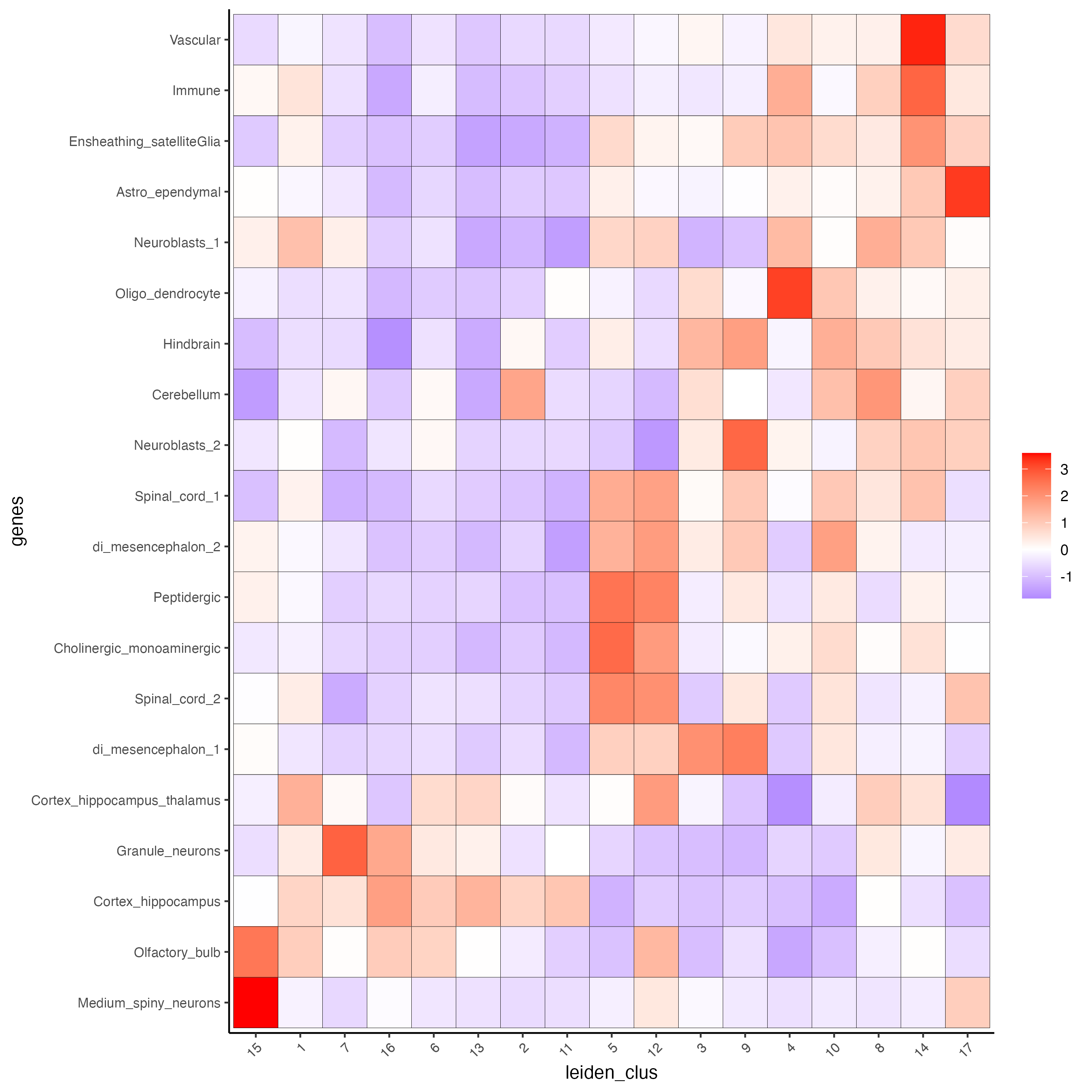```# 1.6 visualizations
spatCellPlot2D(gobject = visium_brain,
spat_enr_names = 'PAGE',
cell_annotation_values = cell_types_PAGE[1:4],
cow_n_col = 2,coord_fix_ratio = 1, point_size = 1.25, show_legend = T)
```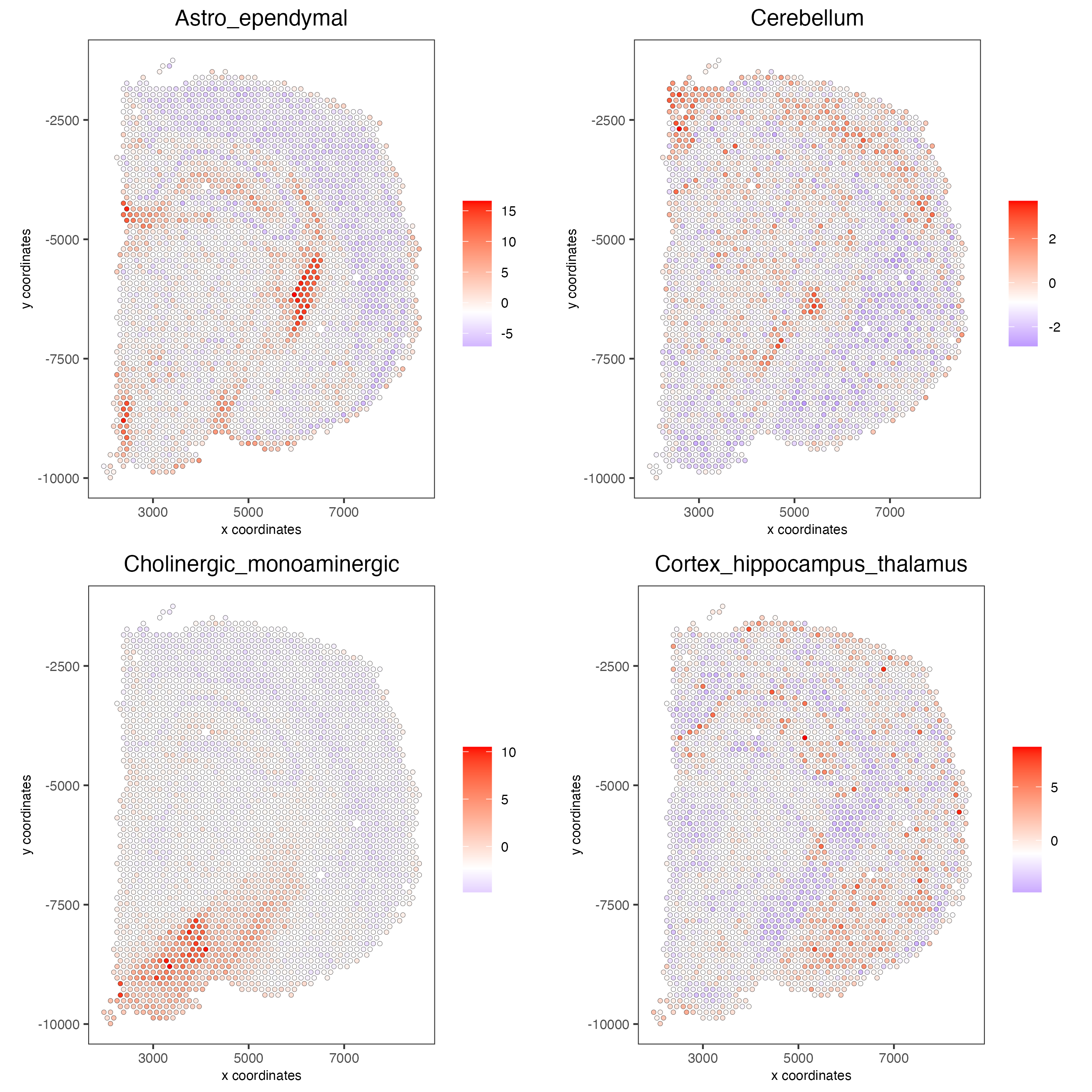```spatDimCellPlot2D(gobject = visium_brain,
spat_enr_names = 'PAGE',
cell_annotation_values = cell_types_PAGE[1:4],
cow_n_col = 1, spat_point_size = 1,
plot_alignment = 'horizontal',
save_param = list(base_width=7, base_height=10))
```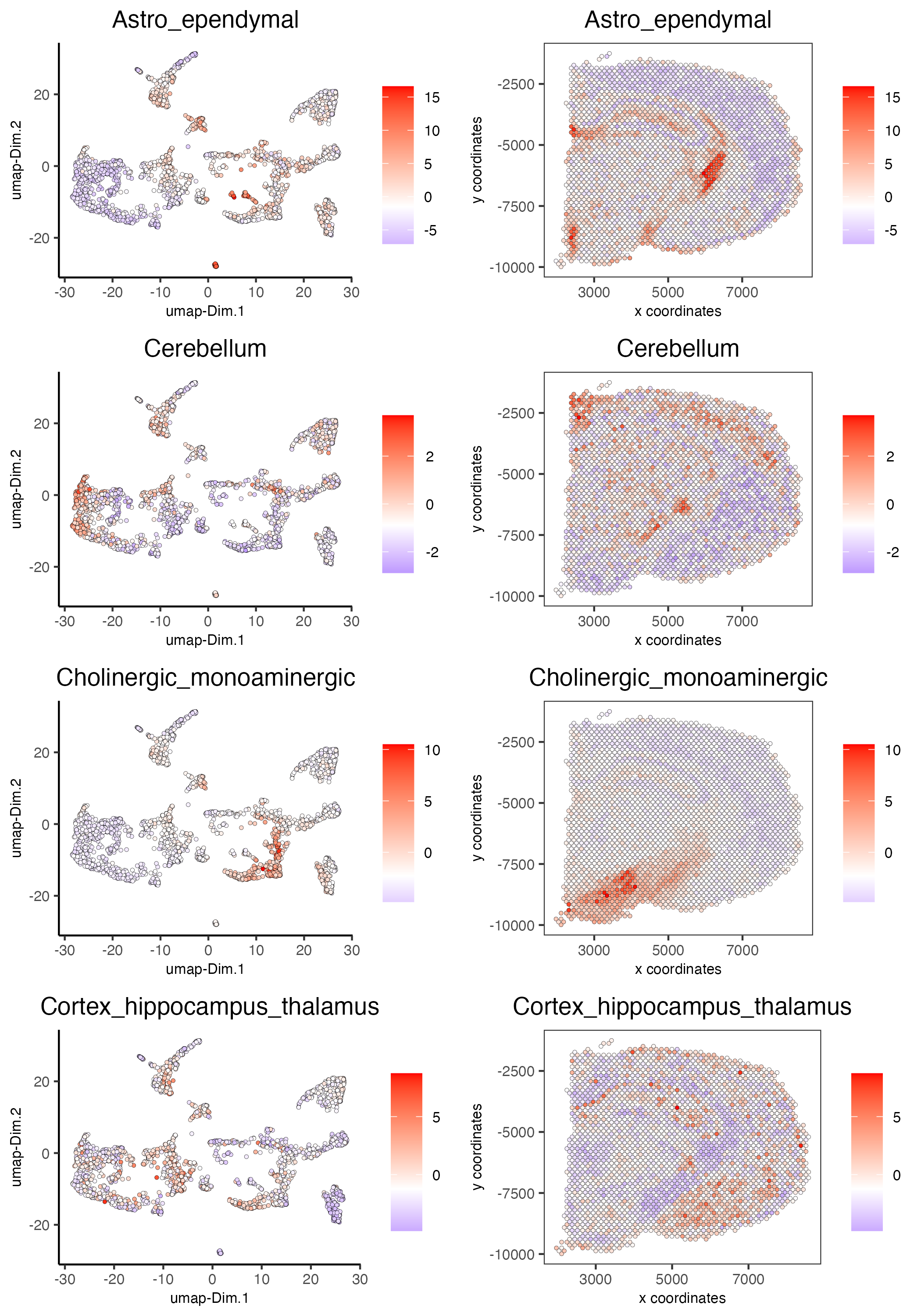### 7.2 HyperGeometric test#

```visium_brain = runHyperGeometricEnrich(gobject = visium_brain,
expression_values = "normalized",
sign_matrix = PAGE_matrix_2)

cell_types_HyperGeometric = colnames(PAGE_matrix_2)
spatCellPlot(gobject = visium_brain,
spat_enr_names = 'hypergeometric',
cell_annotation_values = cell_types_HyperGeometric[1:4],
cow_n_col = 2,coord_fix_ratio = NULL, point_size = 1.75)
```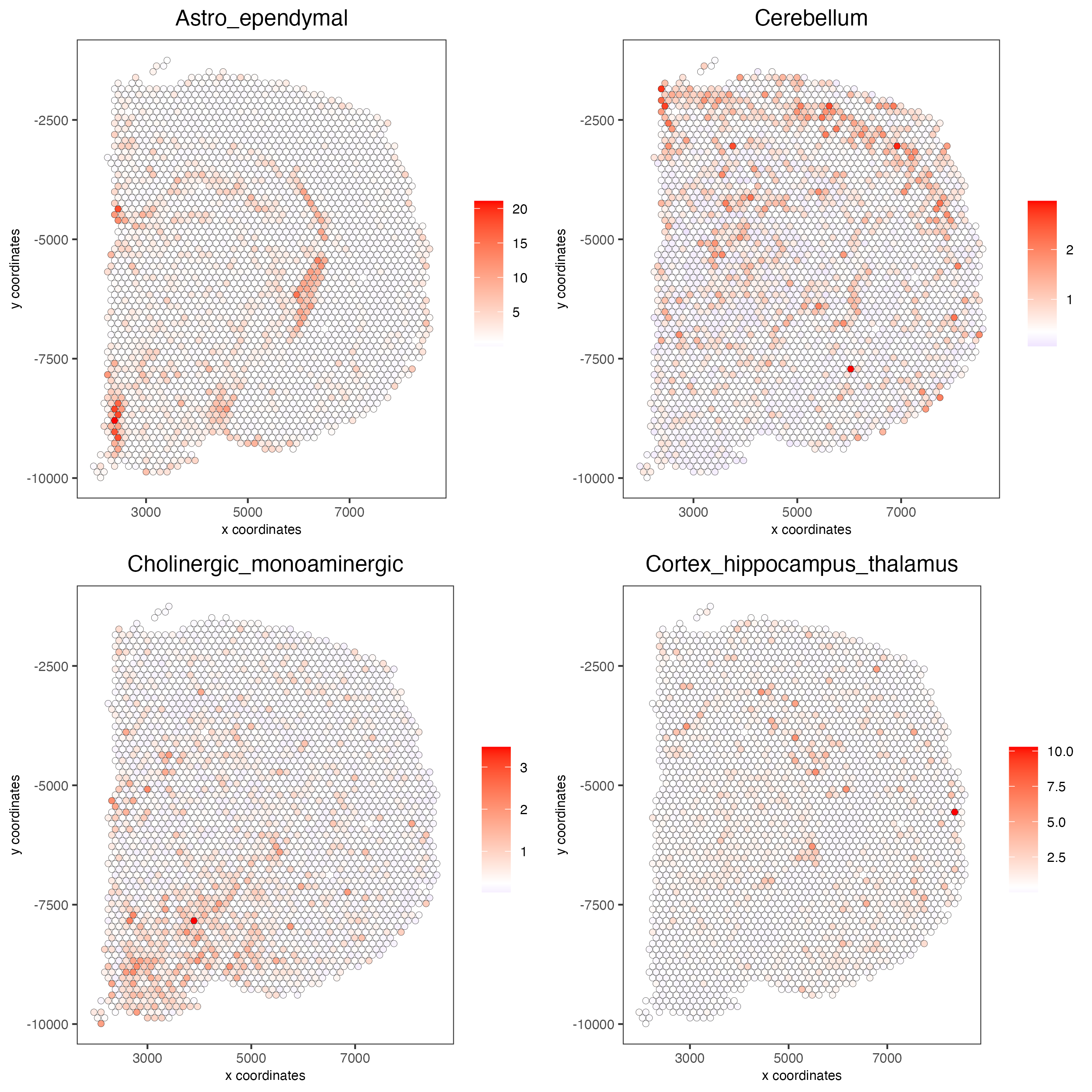### 7.3 Rank Enrichment#

```# Create rank matrix, not that rank matrix is different from PAGE
# A count matrix and a vector for all cell labels will be needed
rank_matrix = makeSignMatrixRank(sc_matrix = get_expression_values(giotto_SC,values = "normalized",output = "matrix"),
visium_brain = runRankEnrich(gobject = visium_brain, sign_matrix = rank_matrix,expression_values = "normalized")

# Plot Rank enrichment result
spatCellPlot2D(gobject = visium_brain,
spat_enr_names = 'rank',
cell_annotation_values = colnames(rank_matrix)[1:4],
cow_n_col = 2,coord_fix_ratio = 1, point_size = 1,
save_param = list(save_name = "spat_enr_Rank_plot"))
```### 7.4 DWLS spatial deconvolution#

```# Create DWLS matrix, not that DWLS matrix is different from PAGE and rank
# A count matrix a vector for a list of gene signatures and a vector for all cell labels will be needed
DWLS_matrix<-makeSignMatrixDWLSfromMatrix(matrix = get_expression_values(giotto_SC,values = "normalized",output = "matrix"),
sign_gene = top_markers\$feats)
visium_brain = runDWLSDeconv(gobject = visium_brain, sign_matrix = DWLS_matrix)

# Plot DWLS deconvolution result
spatCellPlot2D(gobject = visium_brain,
spat_enr_names = 'DWLS',
cow_n_col = 2,coord_fix_ratio = 1, point_size = 1,
save_param = list(save_name = "DWLS_plot"))
```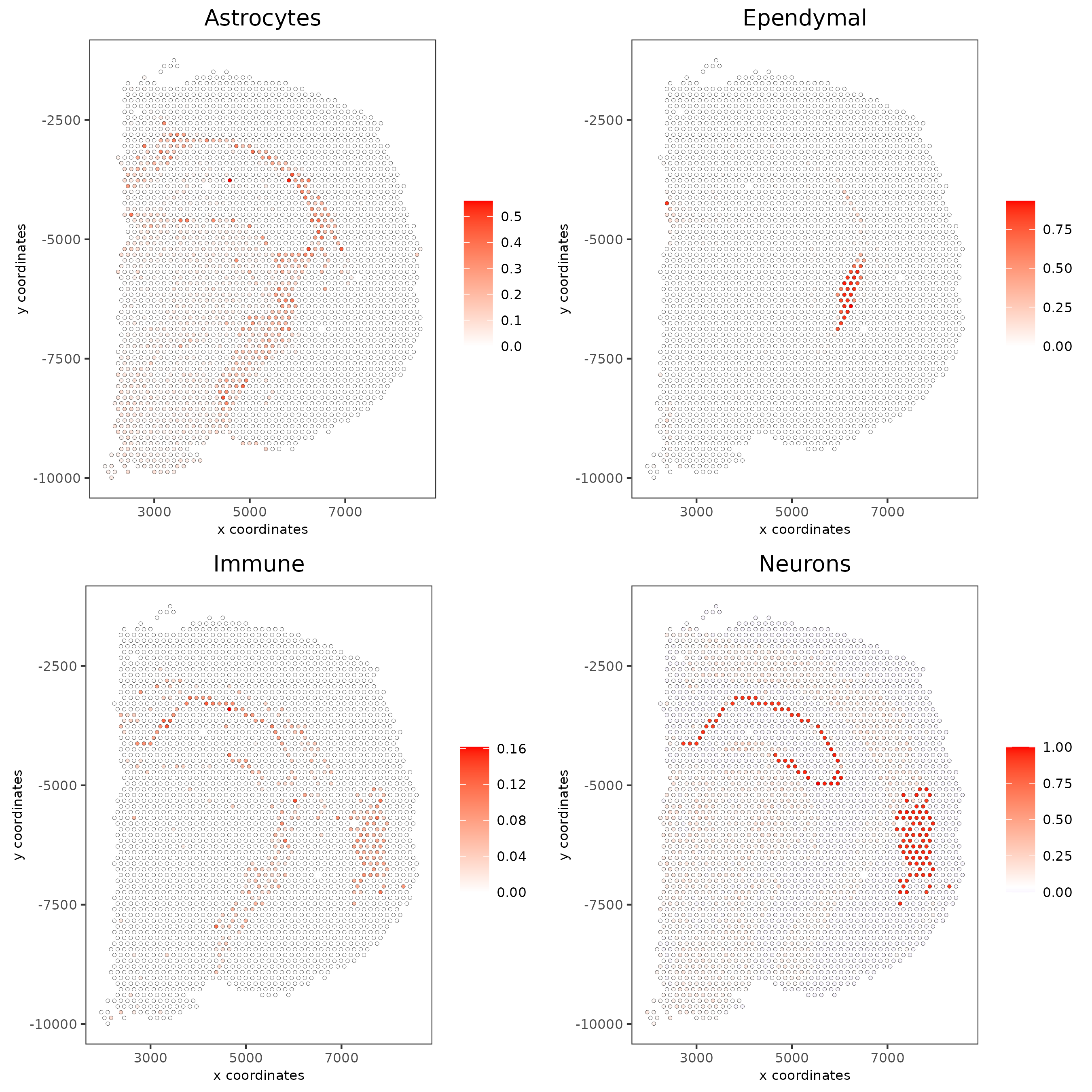```# Plot DWLS deconvolution result with Pie plots
spatDeconvPlot(visium_brain,
show_image = T,
save_param = list(save_name = "spat_DWLS_pie_plot"))
```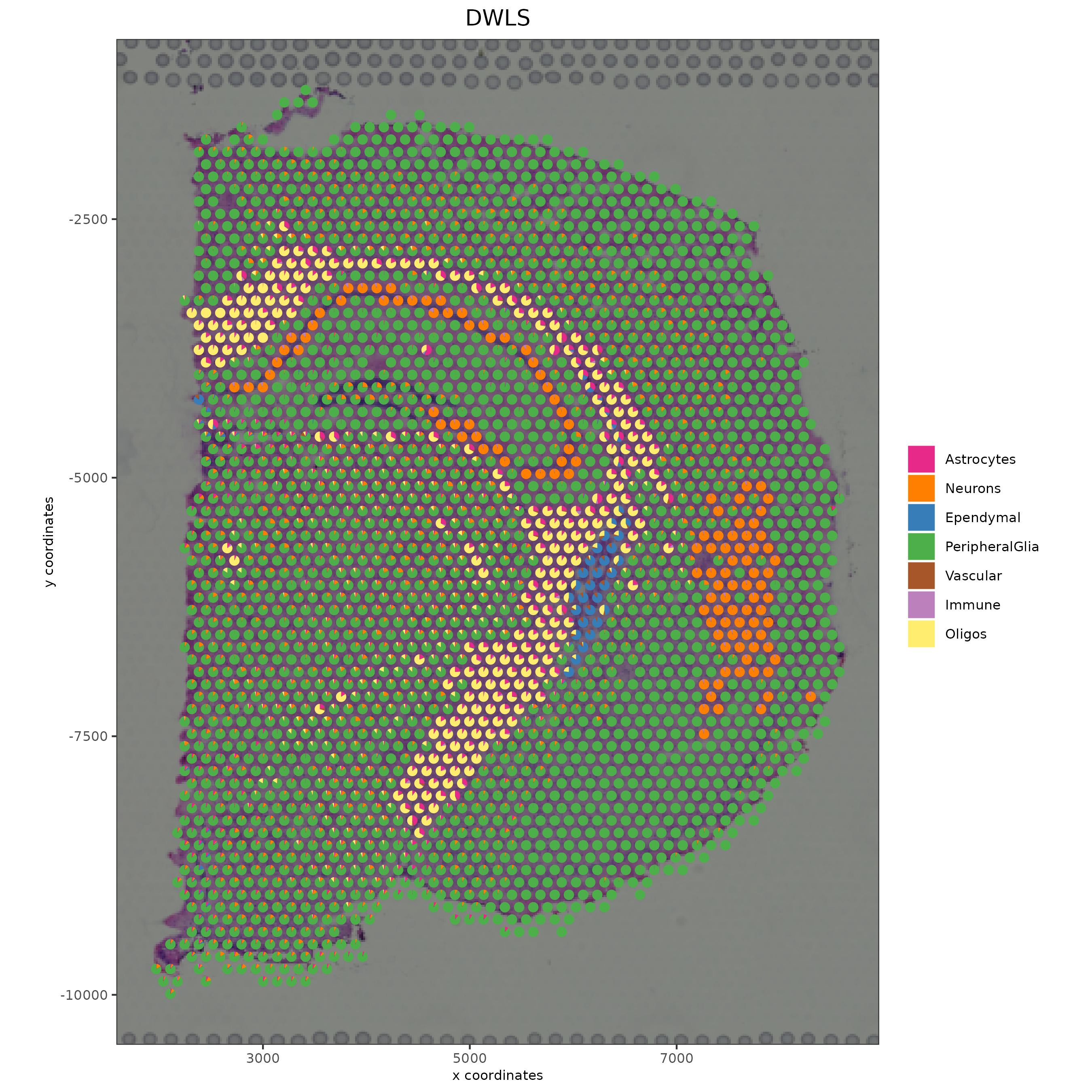## Part 8: Spatial Grid#

```visium_brain <- createSpatialGrid(gobject = visium_brain,
sdimx_stepsize = 400,
sdimy_stepsize = 400,

showGiottoSpatGrids(visium_brain)

spatPlot2D(visium_brain, cell_color = 'leiden_clus', show_grid = T,
grid_color = 'red', spatial_grid_name = 'spatial_grid')
```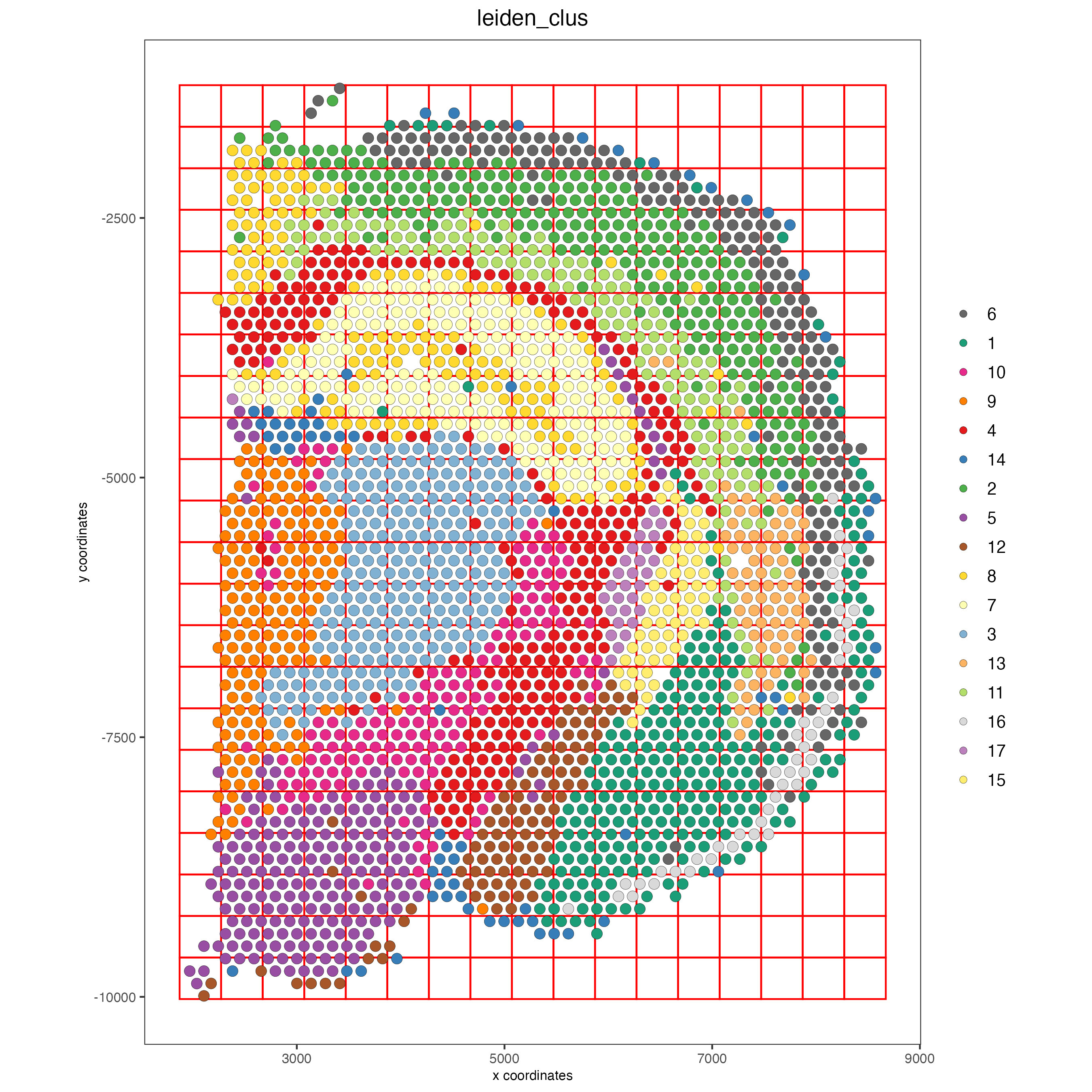## Part 9: spatial network#

```visium_brain <- createSpatialNetwork(gobject = visium_brain,
method = 'kNN', k = 5,
maximum_distance_knn = 400,
name = 'spatial_network')

showGiottoSpatNetworks(visium_brain)

spatPlot2D(gobject = visium_brain,  show_network= T,
network_color = 'blue', spatial_network_name = 'spatial_network')
```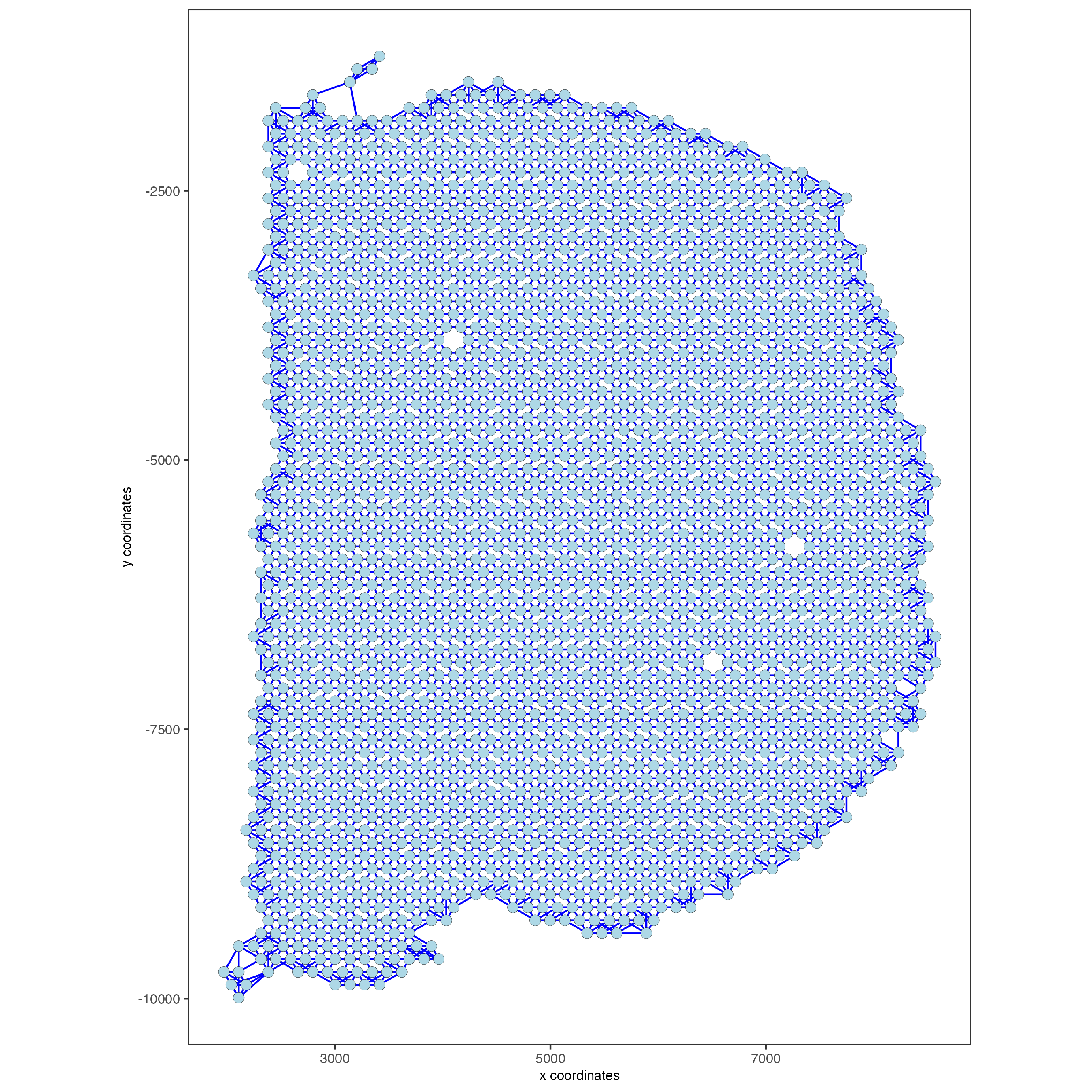## Part 10: Spatial Genes#

```## rank binarization
ranktest = binSpect(visium_brain, bin_method = 'rank',
calc_hub = T, hub_min_int = 5,
spatial_network_name = 'spatial_network')

spatFeatPlot2D(visium_brain, expression_values = 'scaled',
feats = ranktest\$feats[1:6], cow_n_col = 2, point_size = 1.5)
```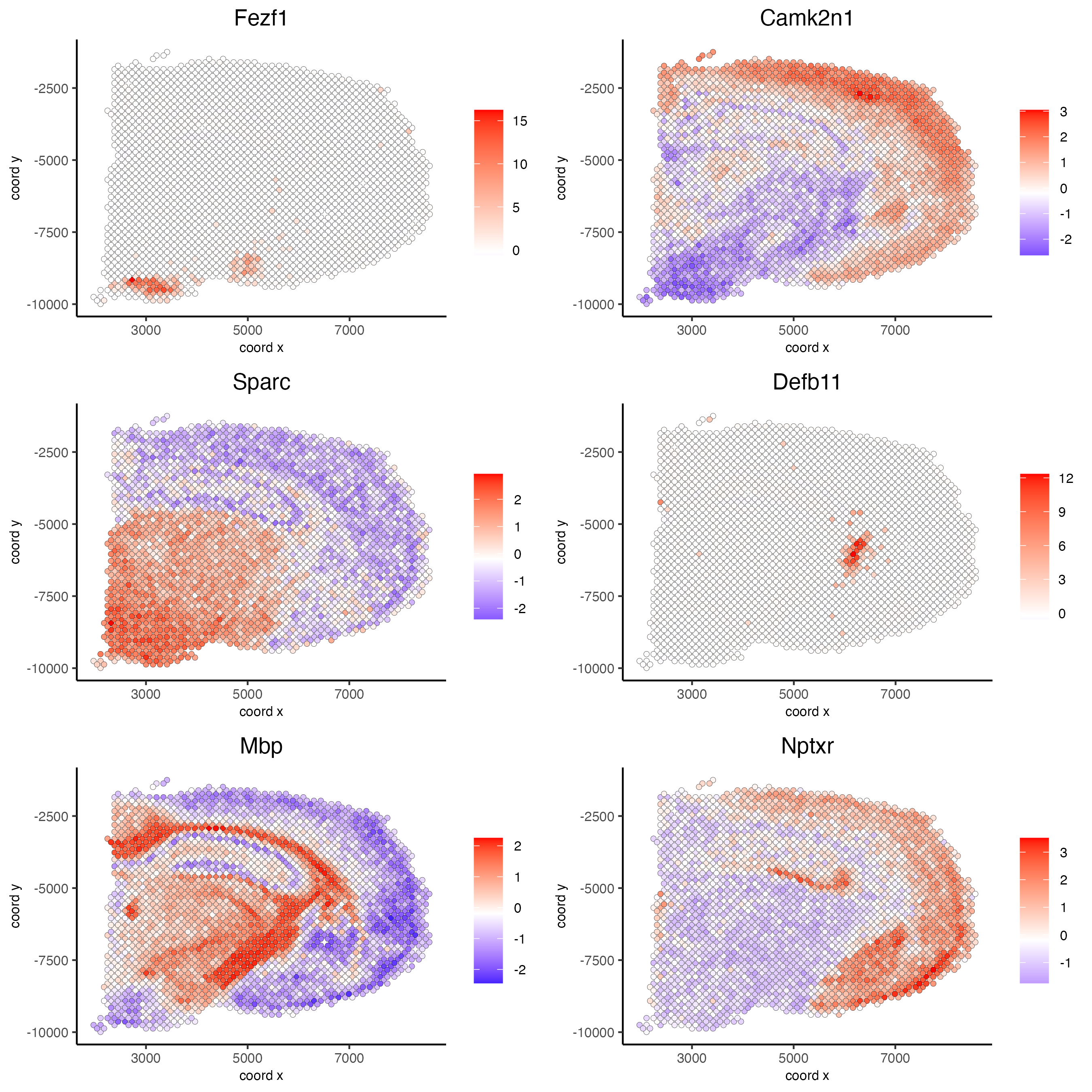## Part 11: Spatial Co-Expression modules#

```# cluster the top 500 spatial genes into 20 clusters
ext_spatial_genes = ranktest[1:1500,]\$feats

# here we use existing detectSpatialCorGenes function to calculate pairwise distances between genes (but set network_smoothing=0 to use default clustering)
spat_cor_netw_DT = detectSpatialCorFeats(visium_brain,
method = 'network',
spatial_network_name = 'spatial_network',
subset_feats = ext_spatial_genes)

# 2. identify most similar spatially correlated genes for one gene
top10_genes = showSpatialCorFeats(spat_cor_netw_DT, feats = 'Ptprn', show_top_feats = 10)

spatFeatPlot2D(visium_brain, expression_values = 'scaled',
feats = top10_genes\$variable[1:4], point_size = 3)
```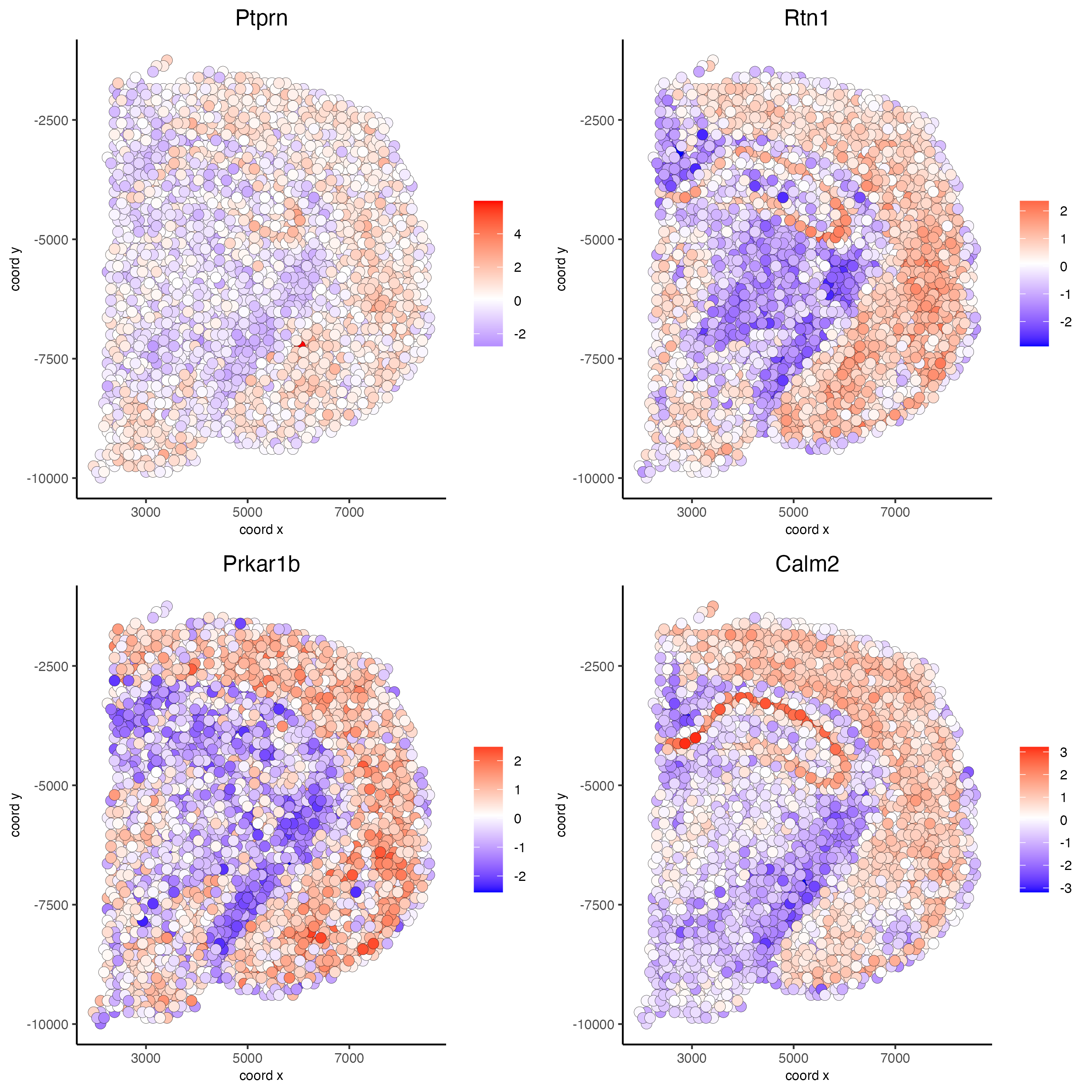```# cluster spatial genes
spat_cor_netw_DT = clusterSpatialCorFeats(spat_cor_netw_DT, name = 'spat_netw_clus', k = 20)

# visualize clusters
heatmSpatialCorFeats(visium_brain,
spatCorObject = spat_cor_netw_DT,
use_clus_name = 'spat_netw_clus',
heatmap_legend_param = list(title = NULL),
save_param = list(base_height = 6, base_width = 8, units = 'cm'))
```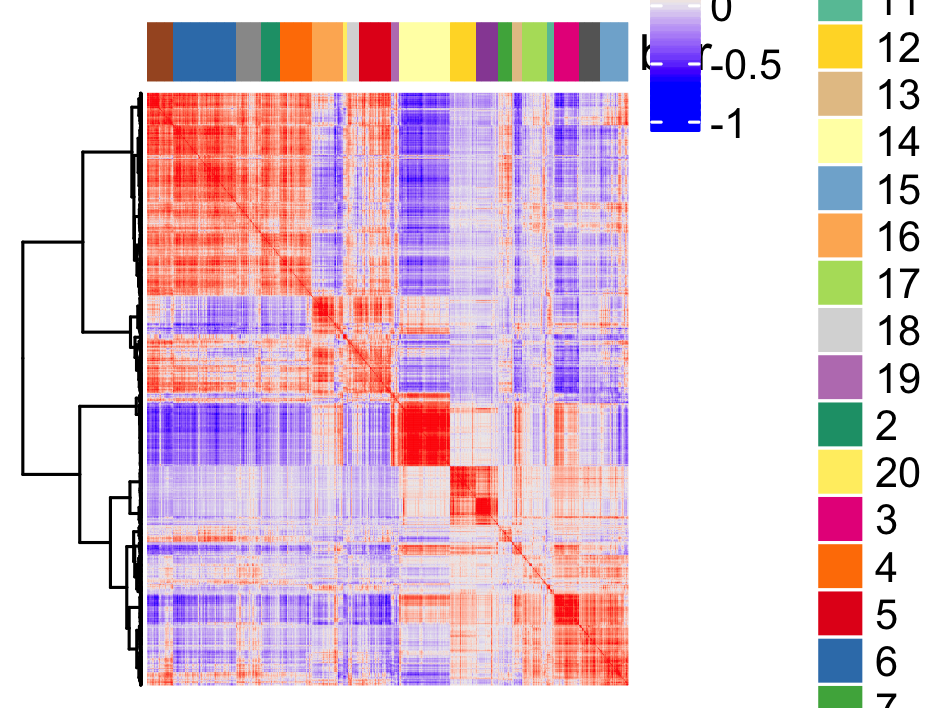```# 4. rank spatial correlated clusters and show genes for selected clusters
netw_ranks = rankSpatialCorGroups(visium_brain,
spatCorObject = spat_cor_netw_DT, use_clus_name = 'spat_netw_clus',
save_param = list(  base_height = 3, base_width = 5))

top_netw_spat_cluster = showSpatialCorFeats(spat_cor_netw_DT, use_clus_name = 'spat_netw_clus',
selected_clusters = 6, show_top_feats = 1)
```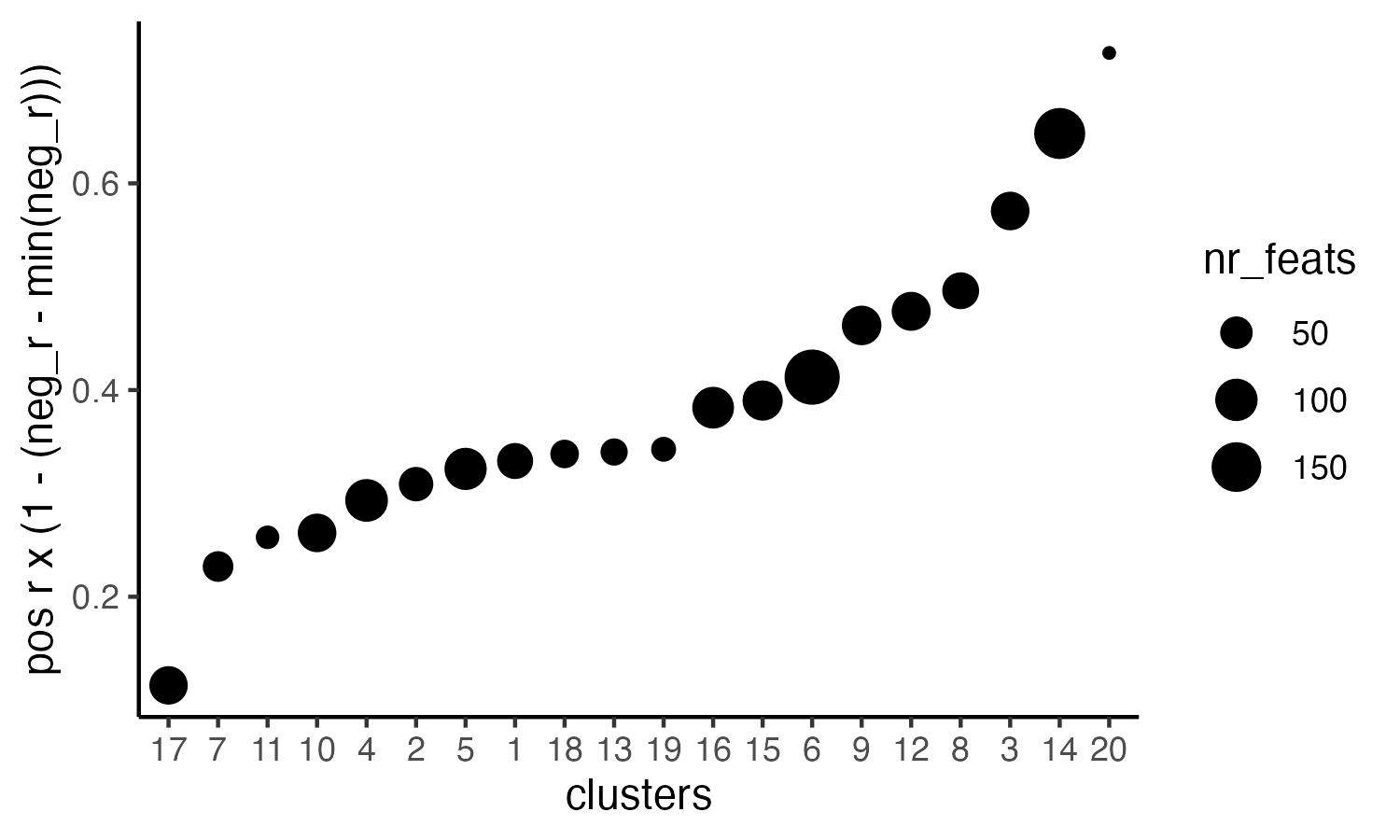```# 5. create metagene enrichment score for clusters
cluster_genes_DT = showSpatialCorFeats(spat_cor_netw_DT, use_clus_name = 'spat_netw_clus', show_top_feats = 1)
cluster_genes = cluster_genes_DT\$clus; names(cluster_genes) = cluster_genes_DT\$feat_ID

visium_brain = createMetafeats(visium_brain, feat_clusters = cluster_genes, name = 'cluster_metagene')

#showGiottoSpatEnrichments(visium_brain)

spatCellPlot(visium_brain,
spat_enr_names = 'cluster_metagene',
cell_annotation_values = netw_ranks\$clusters,
point_size = 1, cow_n_col = 5, save_param = list(base_width = 15))
```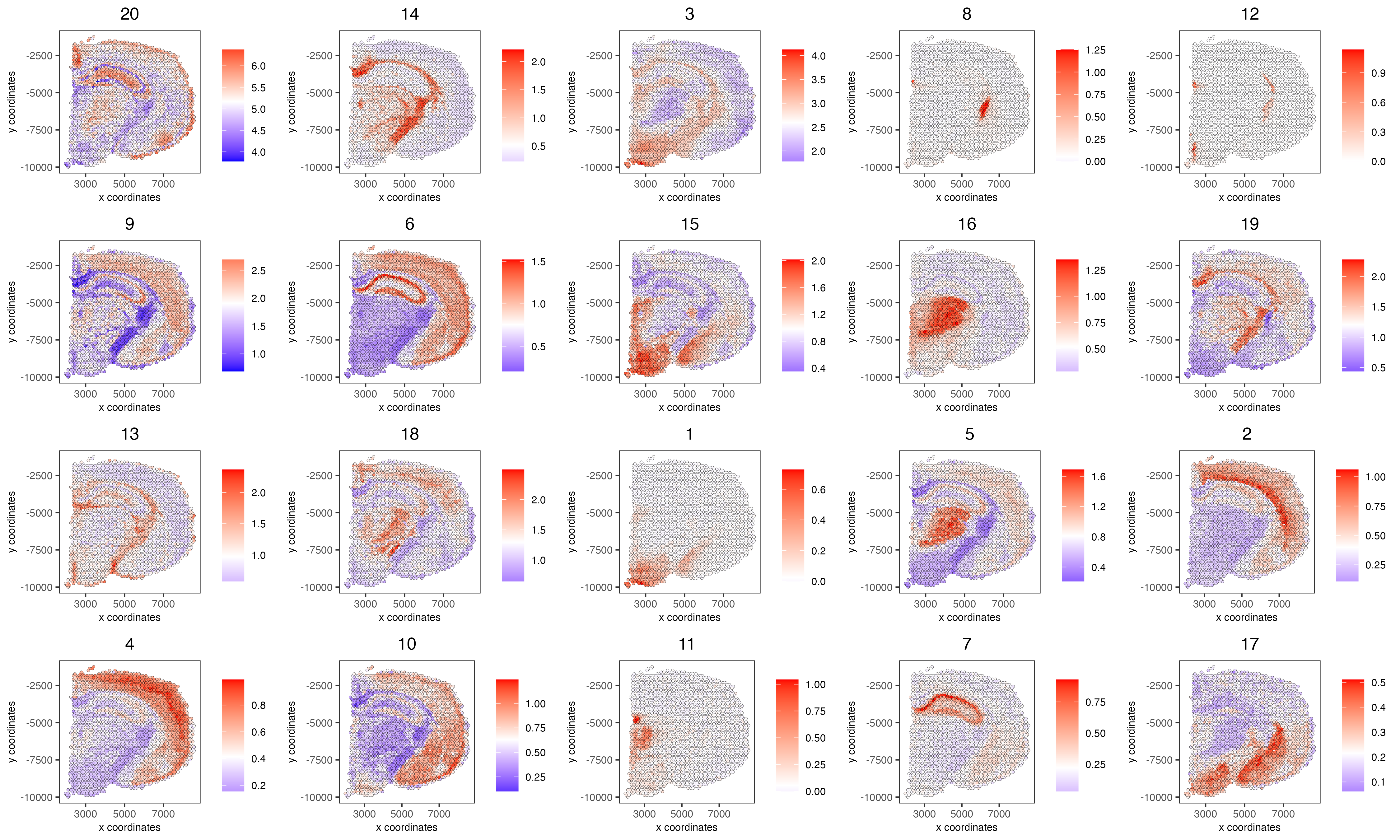## Part 12: Spatially informed clusters#

```# top 30 genes per spatial co-expression cluster
table(spat_cor_netw_DT\$cor_clusters\$spat_netw_clus)
coexpr_dt = data.table::data.table(genes = names(spat_cor_netw_DT\$cor_clusters\$spat_netw_clus),
cluster = spat_cor_netw_DT\$cor_clusters\$spat_netw_clus)
data.table::setorder(coexpr_dt, cluster)
top30_coexpr_dt = coexpr_dt[, head(.SD, 30) , by = cluster]
my_spatial_genes <- top30_coexpr_dt\$genes

visium_brain <- runPCA(gobject = visium_brain,
feats_to_use = my_spatial_genes,
name = 'custom_pca')
visium_brain <- runUMAP(visium_brain, dim_reduction_name = 'custom_pca', dimensions_to_use = 1:20,
name = 'custom_umap')
visium_brain <- createNearestNetwork(gobject = visium_brain,
dim_reduction_name = 'custom_pca',
dimensions_to_use = 1:20, k = 5,
name = 'custom_NN')
visium_brain <- doLeidenCluster(gobject = visium_brain, network_name = 'custom_NN',
resolution = 0.15, n_iterations = 1000,
name = 'custom_leiden')

cell_clusters = unique(cell_meta\$custom_leiden)

selected_colors = getDistinctColors(length(cell_clusters))
names(selected_colors) = cell_clusters

spatPlot2D(visium_brain, cell_color = 'custom_leiden', cell_color_code = selected_colors, coord_fix_ratio = 1)
```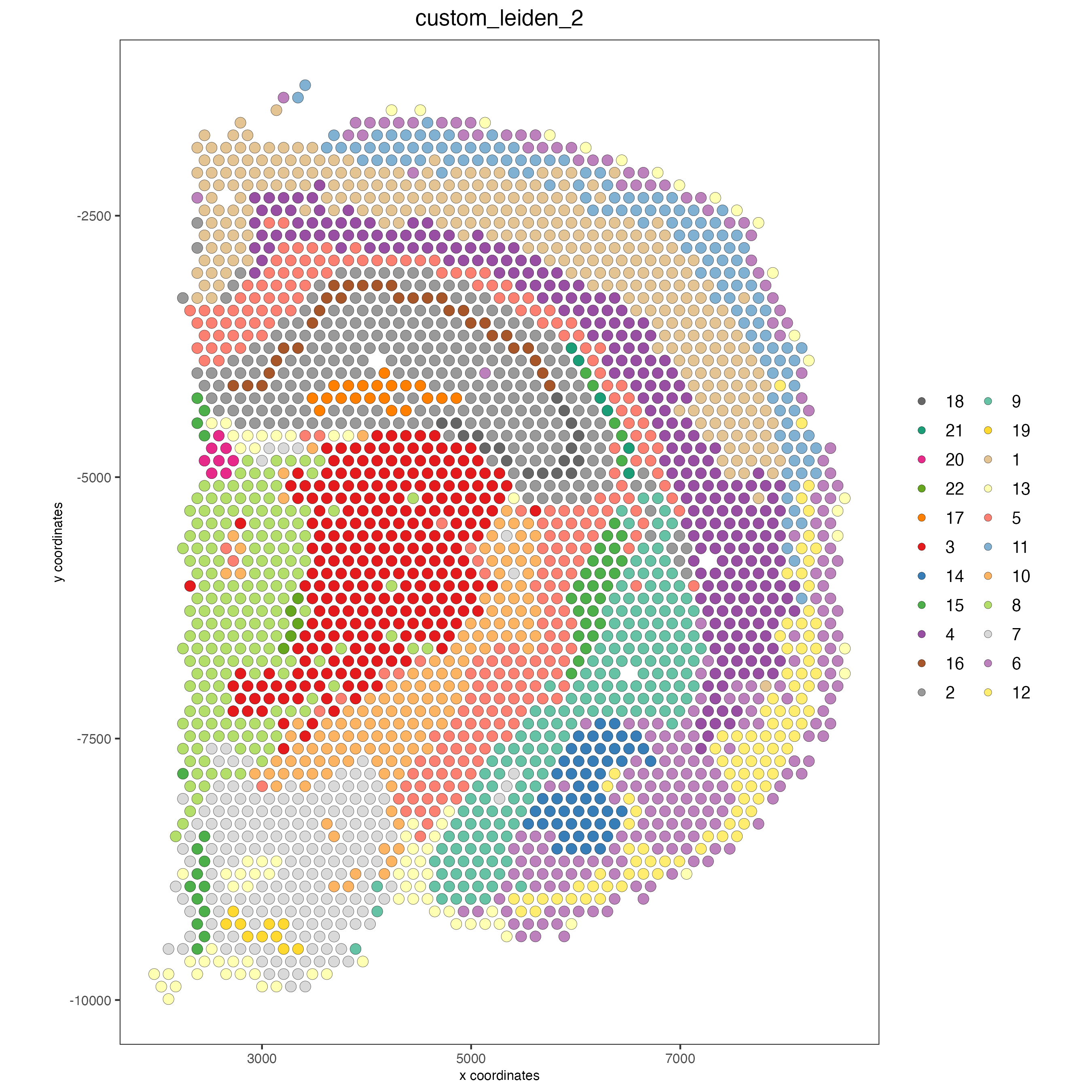```plotUMAP(gobject = visium_brain, cell_color = 'custom_leiden', cell_color_code = selected_colors, point_size = 1.5)
```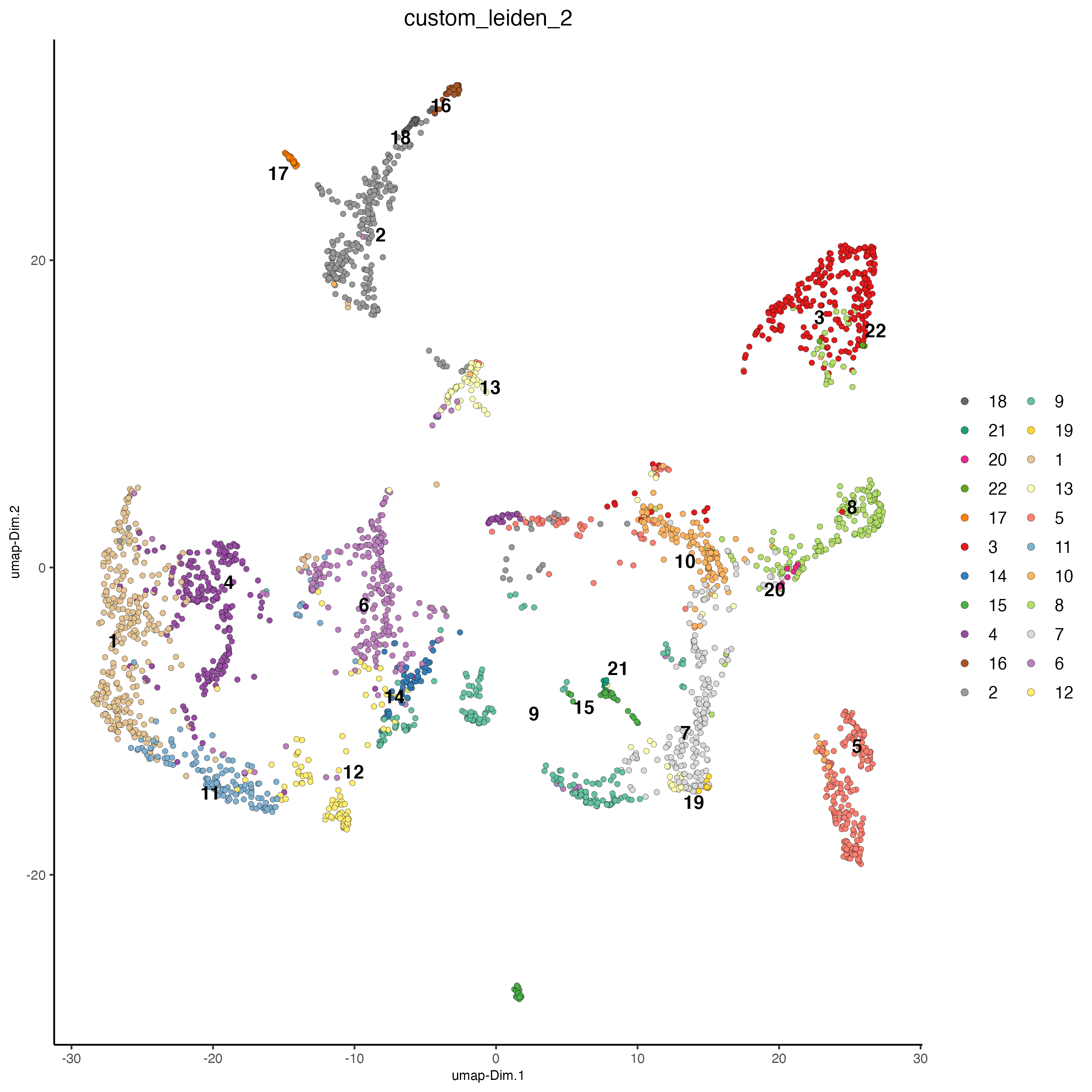## Part 13: Spatial domains with HMRF#

```# do HMRF with different betas on top 30 genes per spatial co-expression module
hmrf_folder = paste0(results_folder,'/','11_HMRF/')
if(!file.exists(hmrf_folder)) dir.create(hmrf_folder, recursive = T)

HMRF_spatial_genes = doHMRF(gobject = visium_brain,
expression_values = 'scaled',
spatial_genes = my_spatial_genes, k = 20,
spatial_network_name="spatial_network",
betas = c(0, 10, 5),
output_folder = paste0(hmrf_folder, '/', 'Spatial_genes/SG_topgenes_k20_scaled'))

visium_brain = addHMRF(gobject = visium_brain, HMRFoutput = HMRF_spatial_genes,
k = 20, betas_to_add = c(0, 10, 20, 30, 40),
hmrf_name = 'HMRF')

spatPlot2D(gobject = visium_brain, cell_color = 'HMRF_k20_b.40')
```